# Concise Selina Solutions for Class 9 Maths Chapter 8-Logarithms

The Selina solutions for the questions given in Chapter 8, Logarithms, of the Class 9 Selina textbooks are available here. In this chapter students learn about the topic of Logarithms in detail. Students can easily score full marks in the exams by solving all the questions present in the Selina textbook.

The Class 9 Selina solutions maths are very easy to understand. These solutions cover all the exercise questions included in the book and are according to the syllabus prescribed by the ICSE or CISCE. Here, the PDF of the Class 9 maths Chapter 8 Selina solutions is available which can be downloaded as well as viewed online. Students can also avail these Selina solutions and download it for free to practice them offline as well.

### Access Answers of Maths Selina Class 9 Chapter 8- Logarithms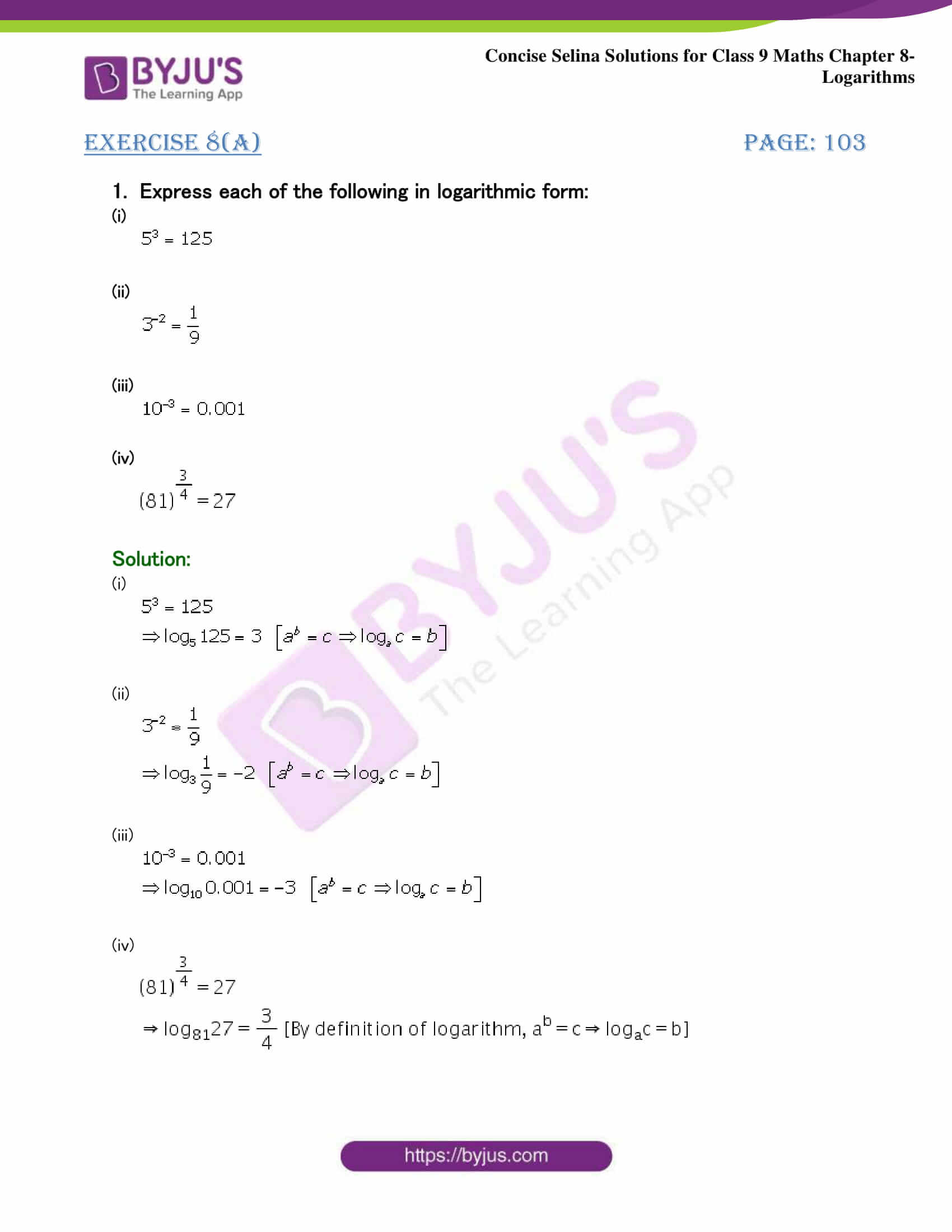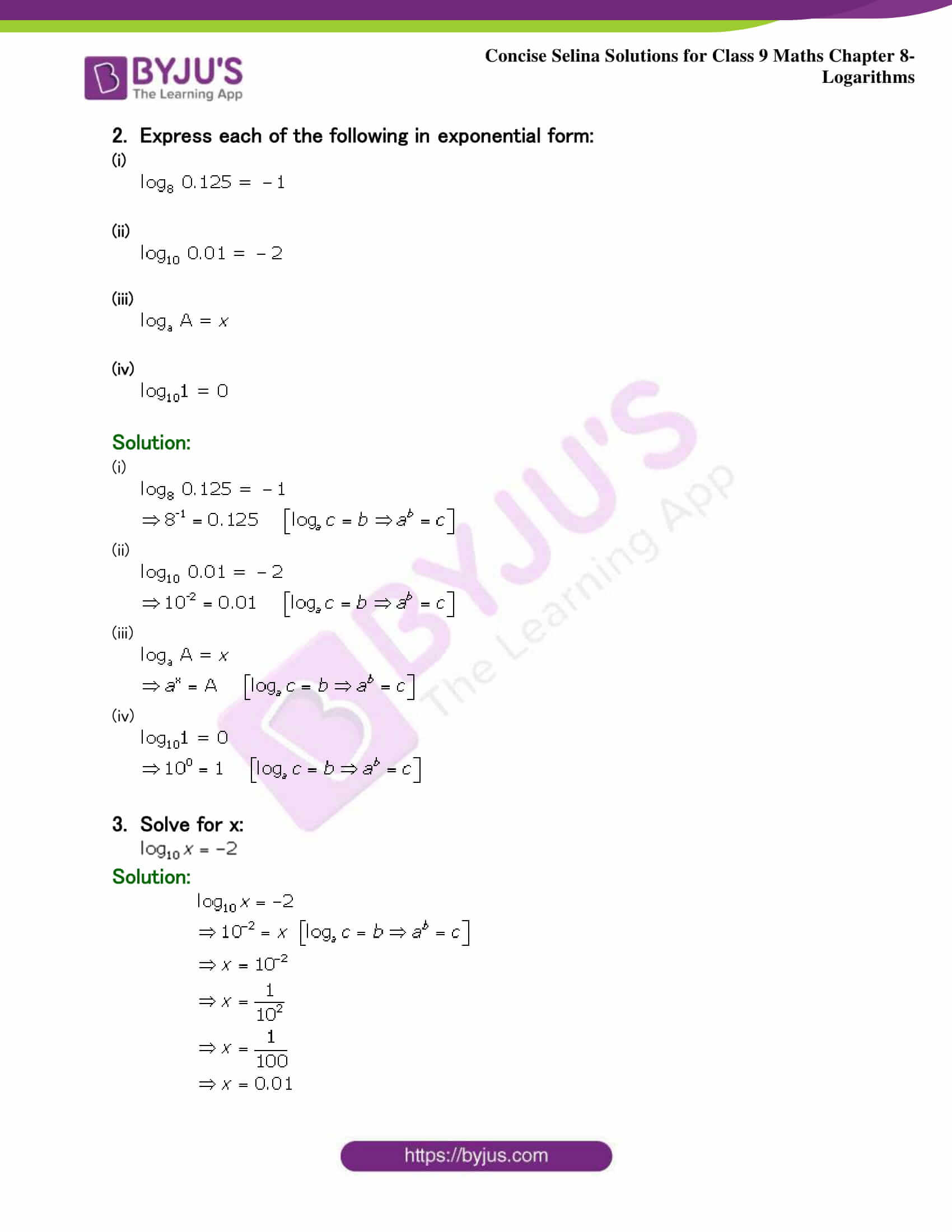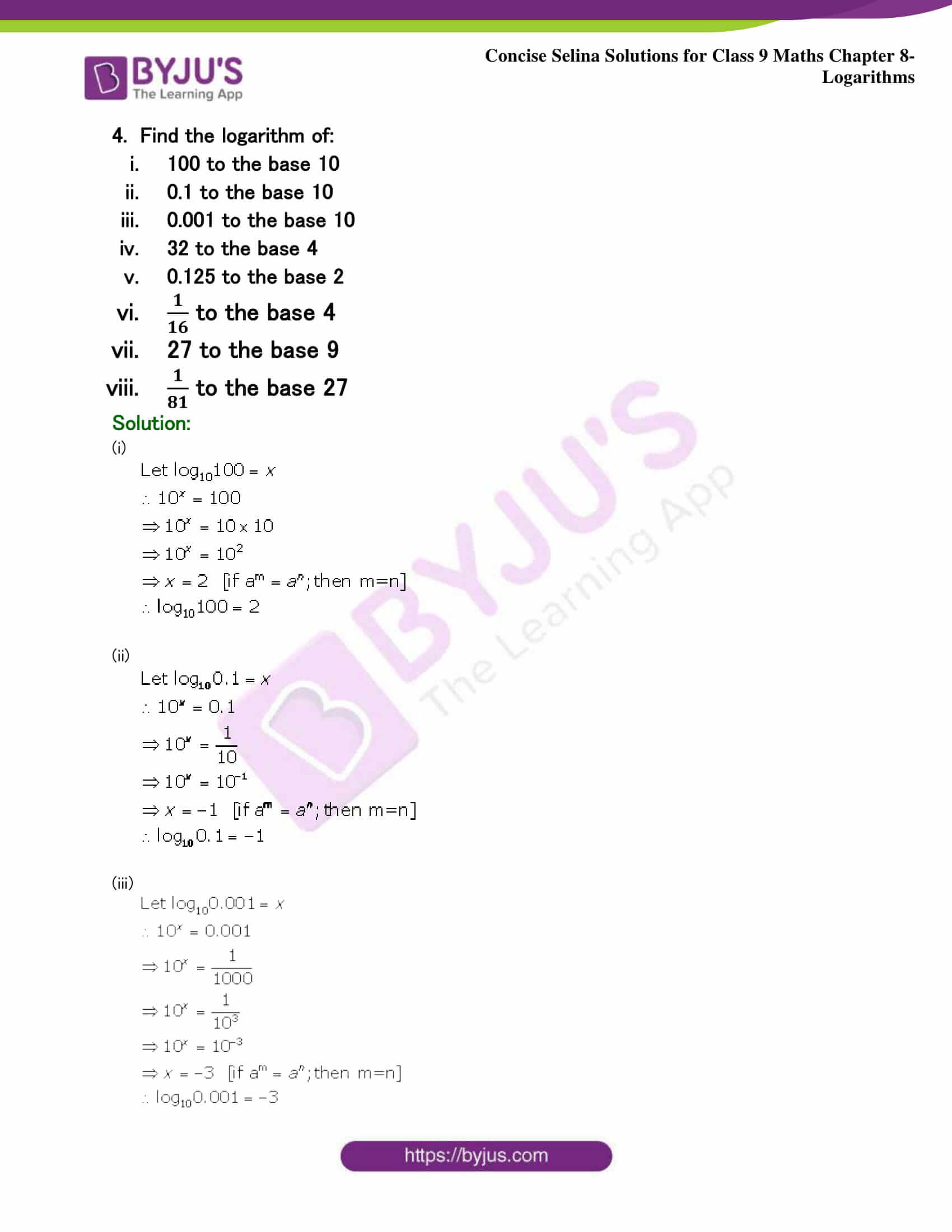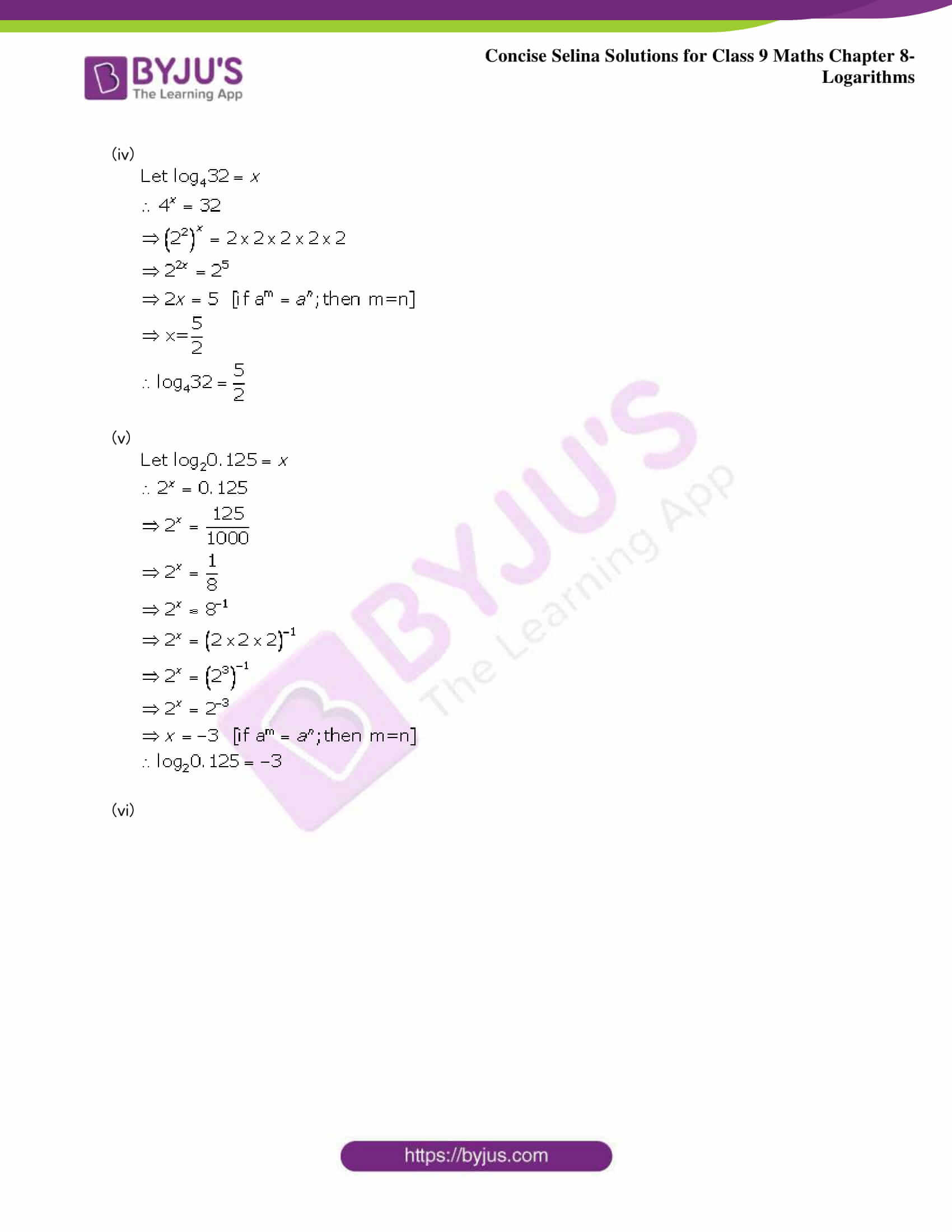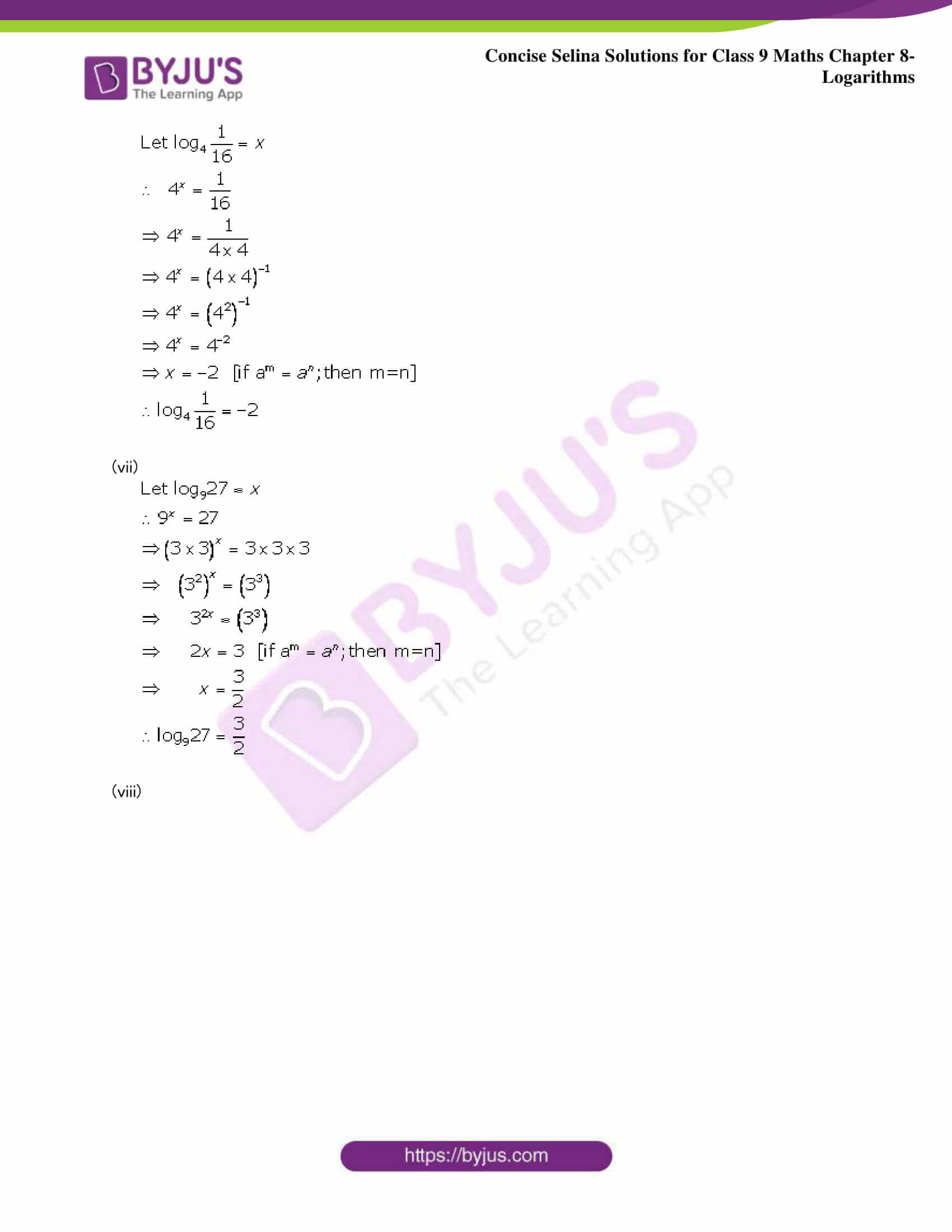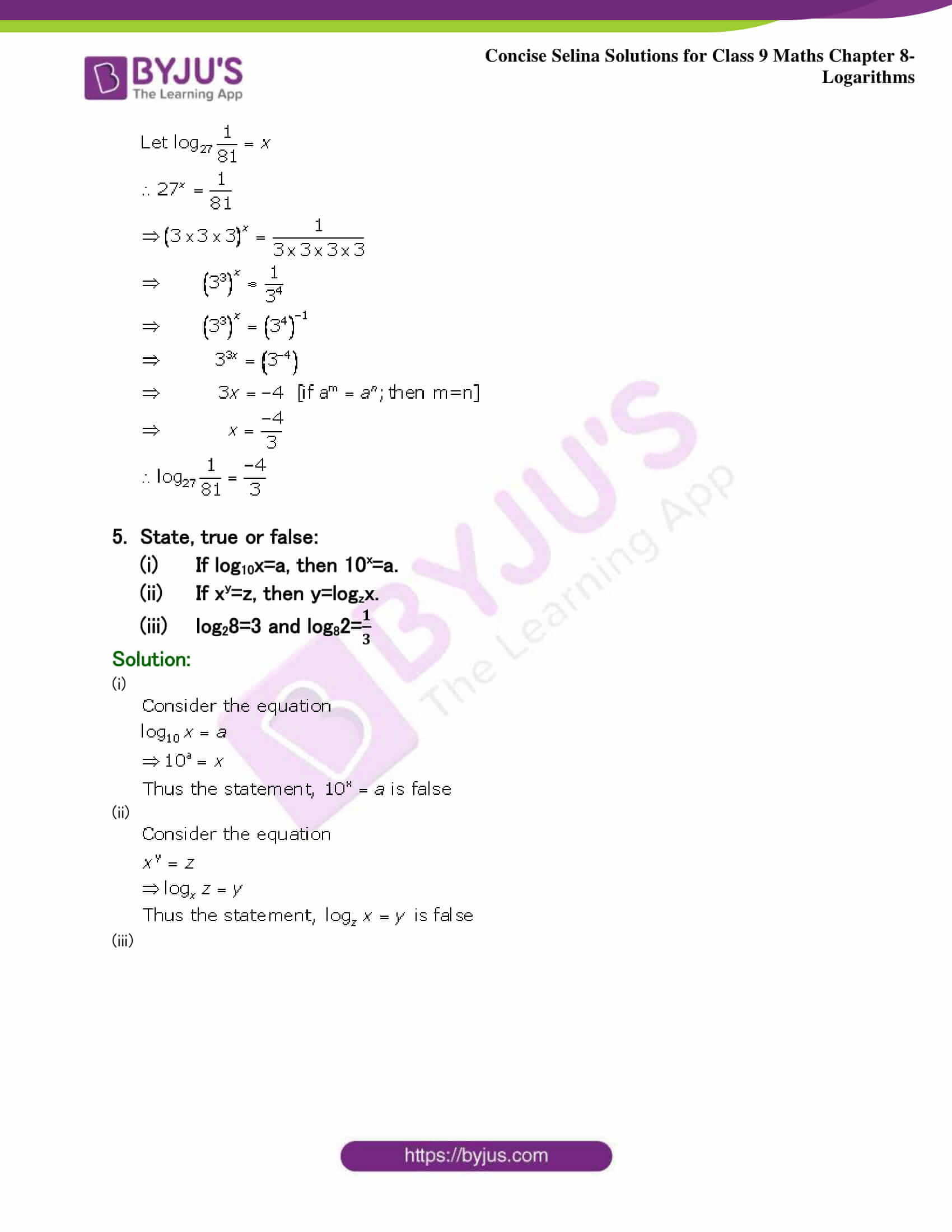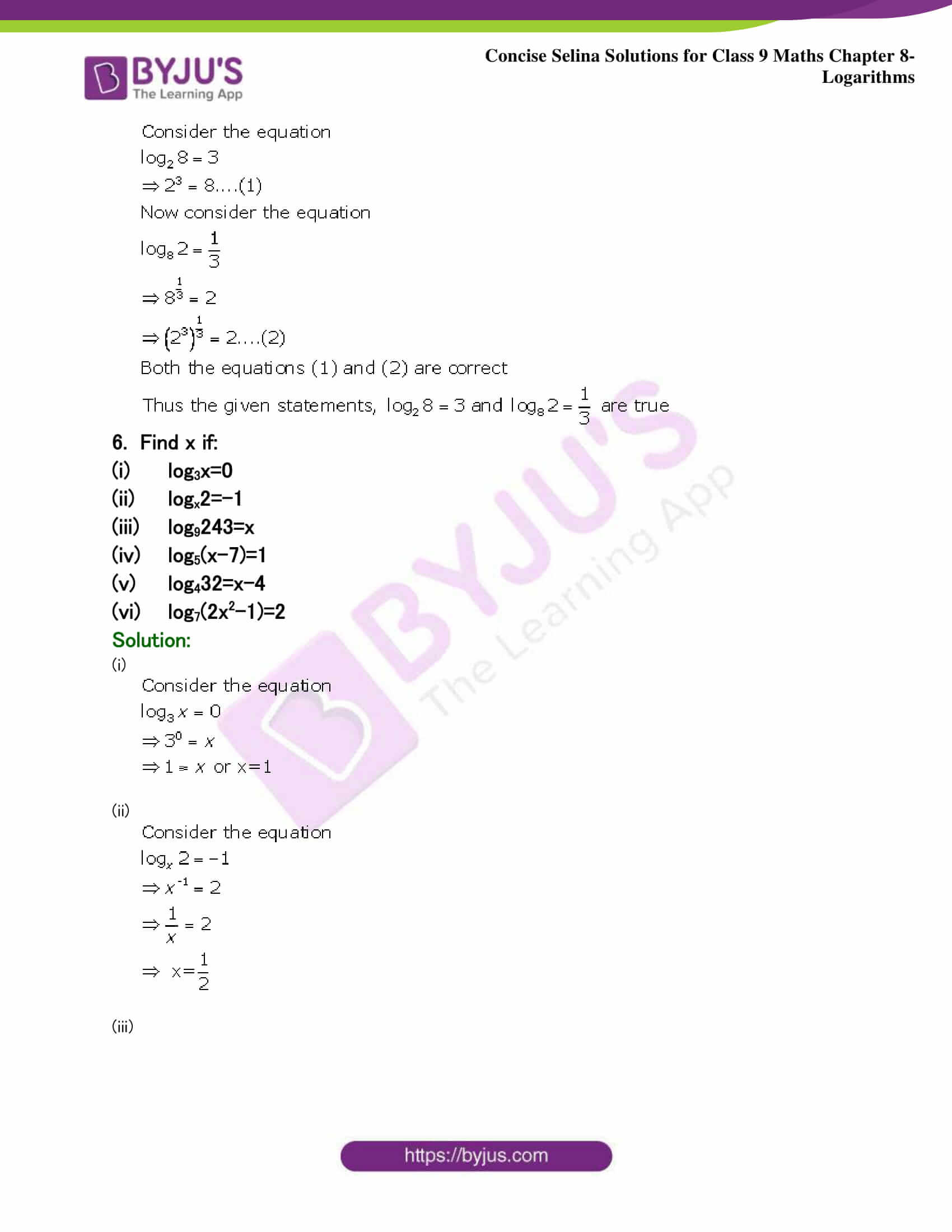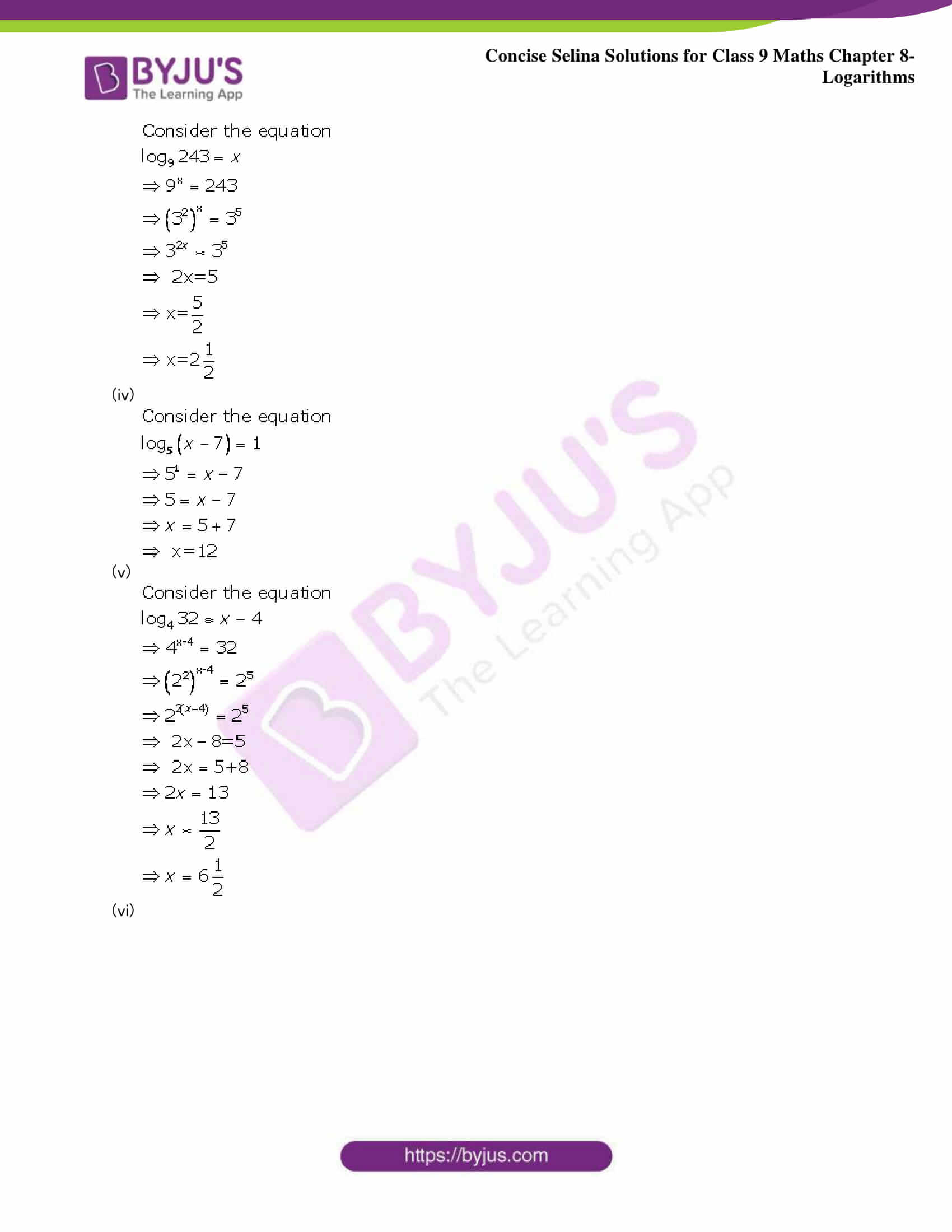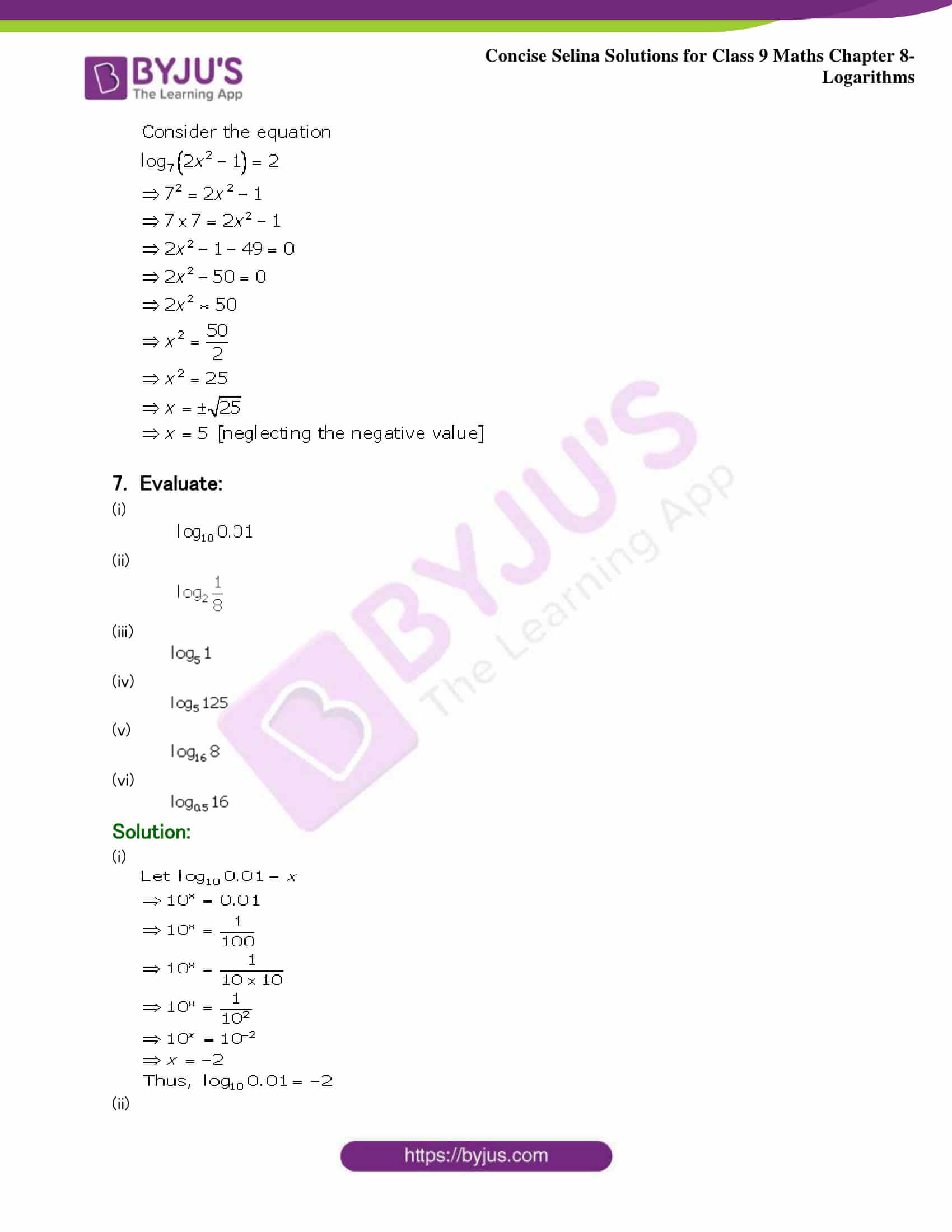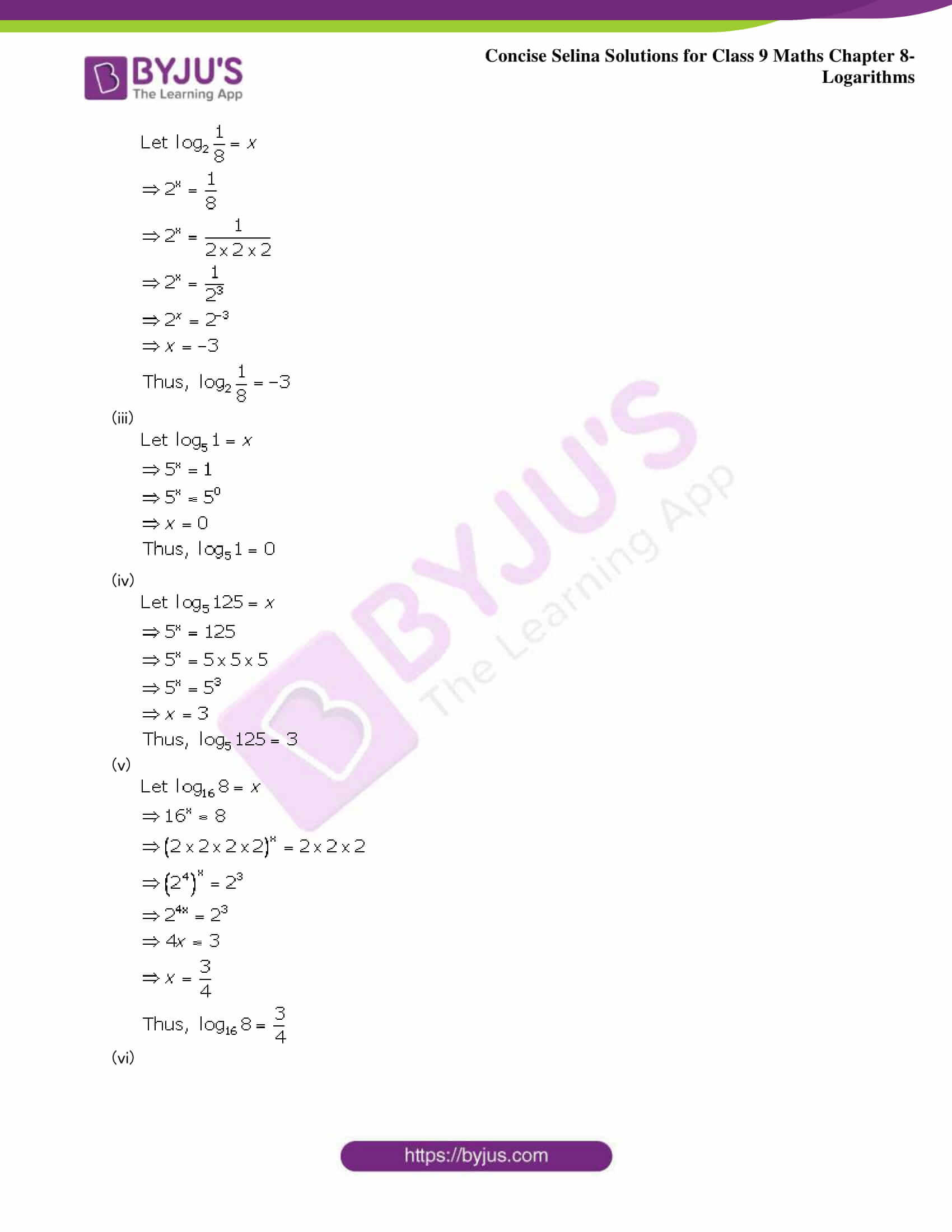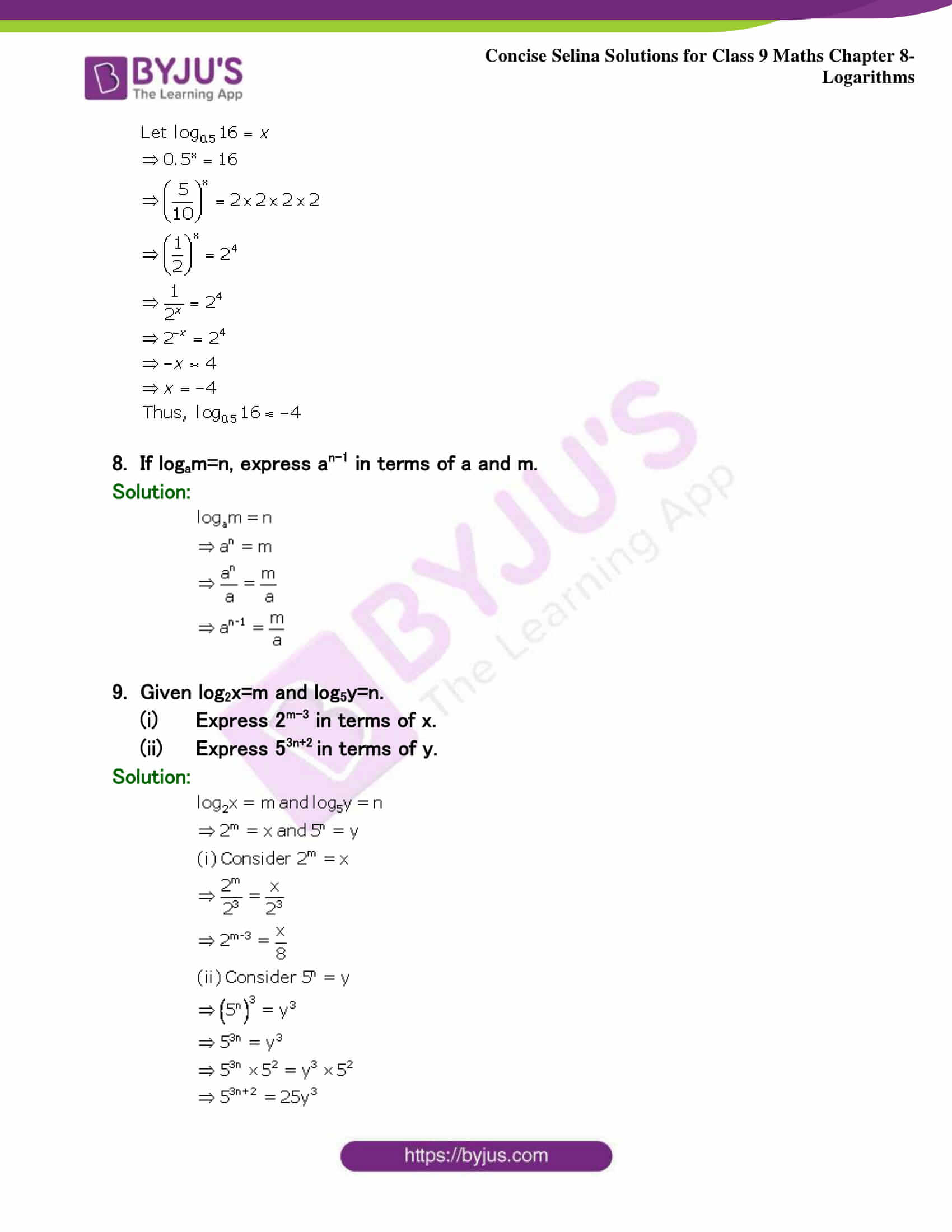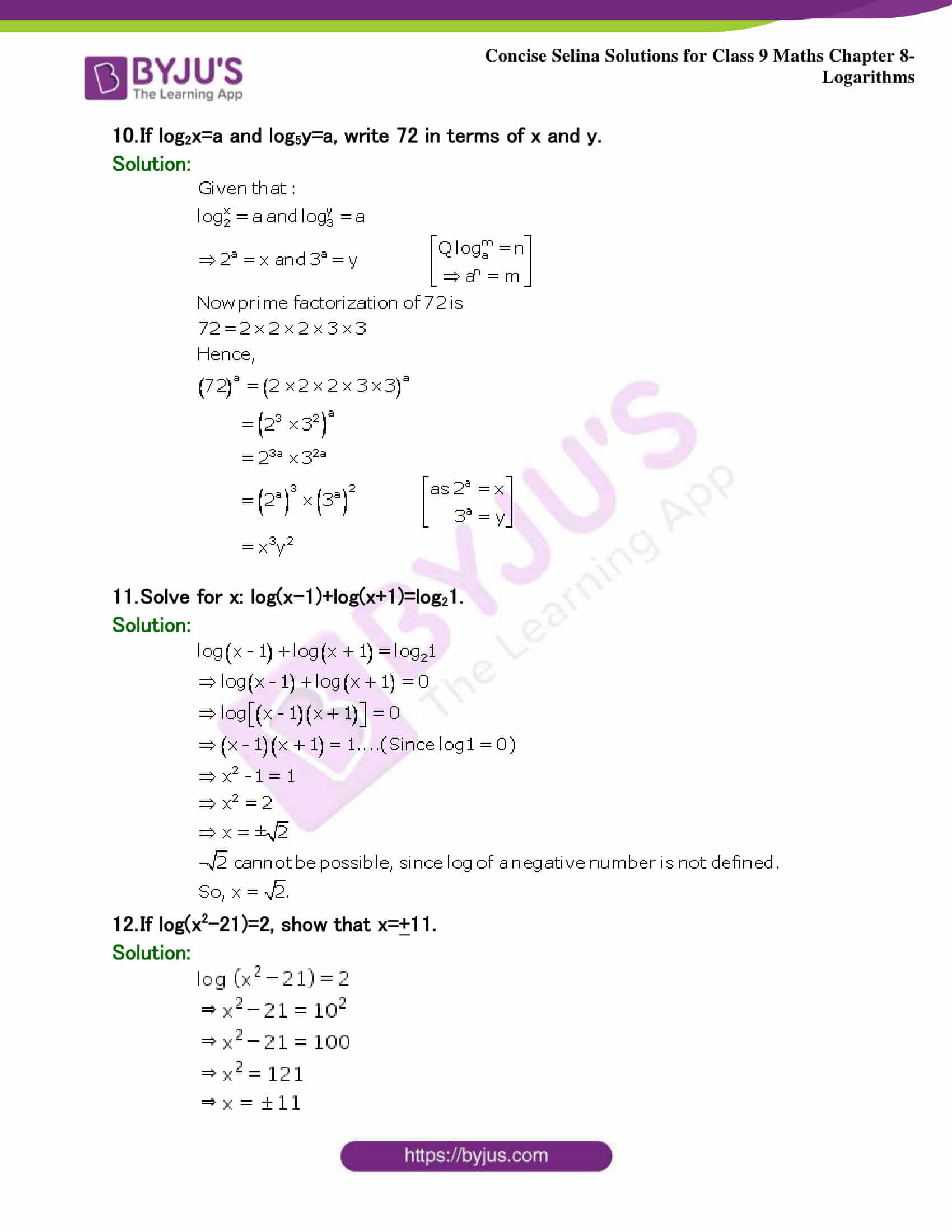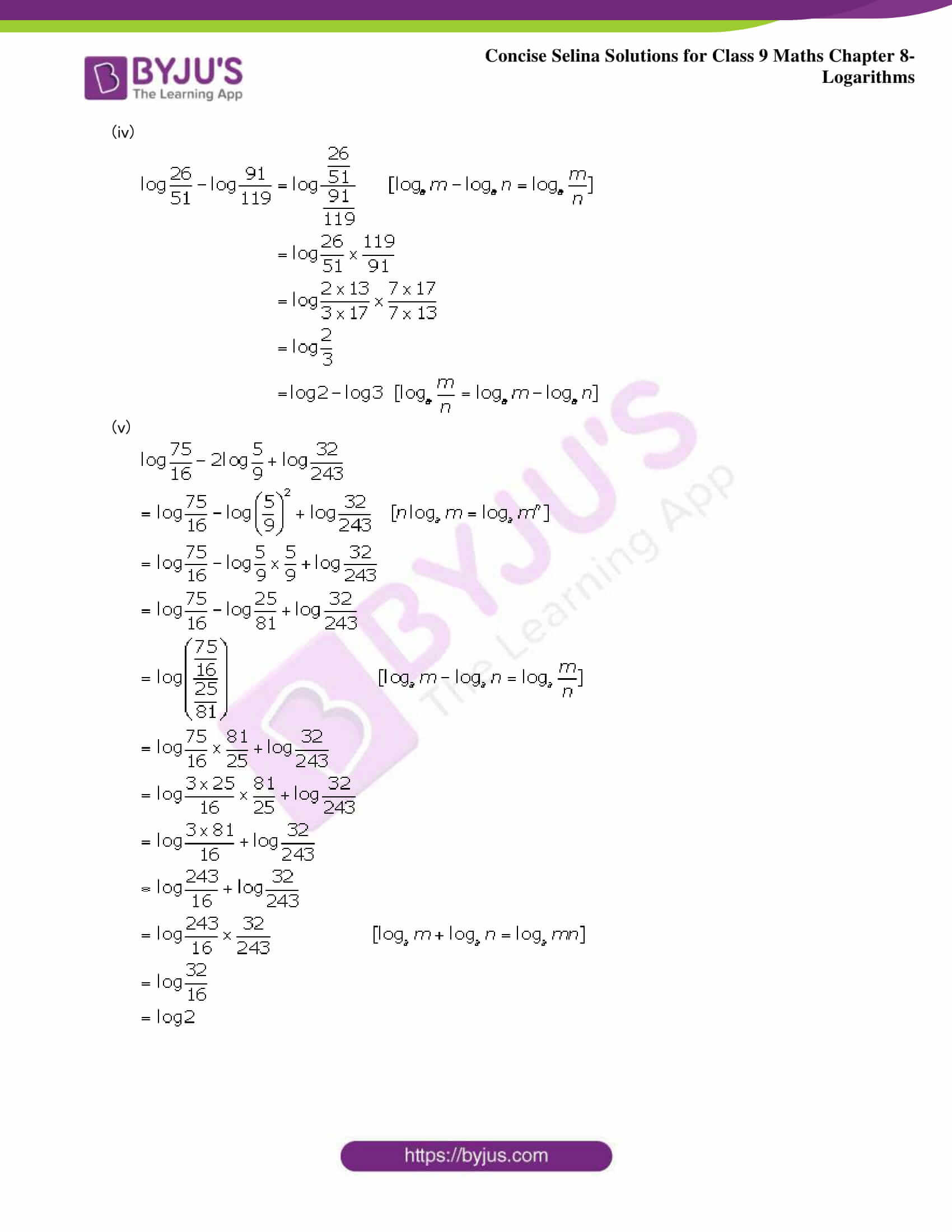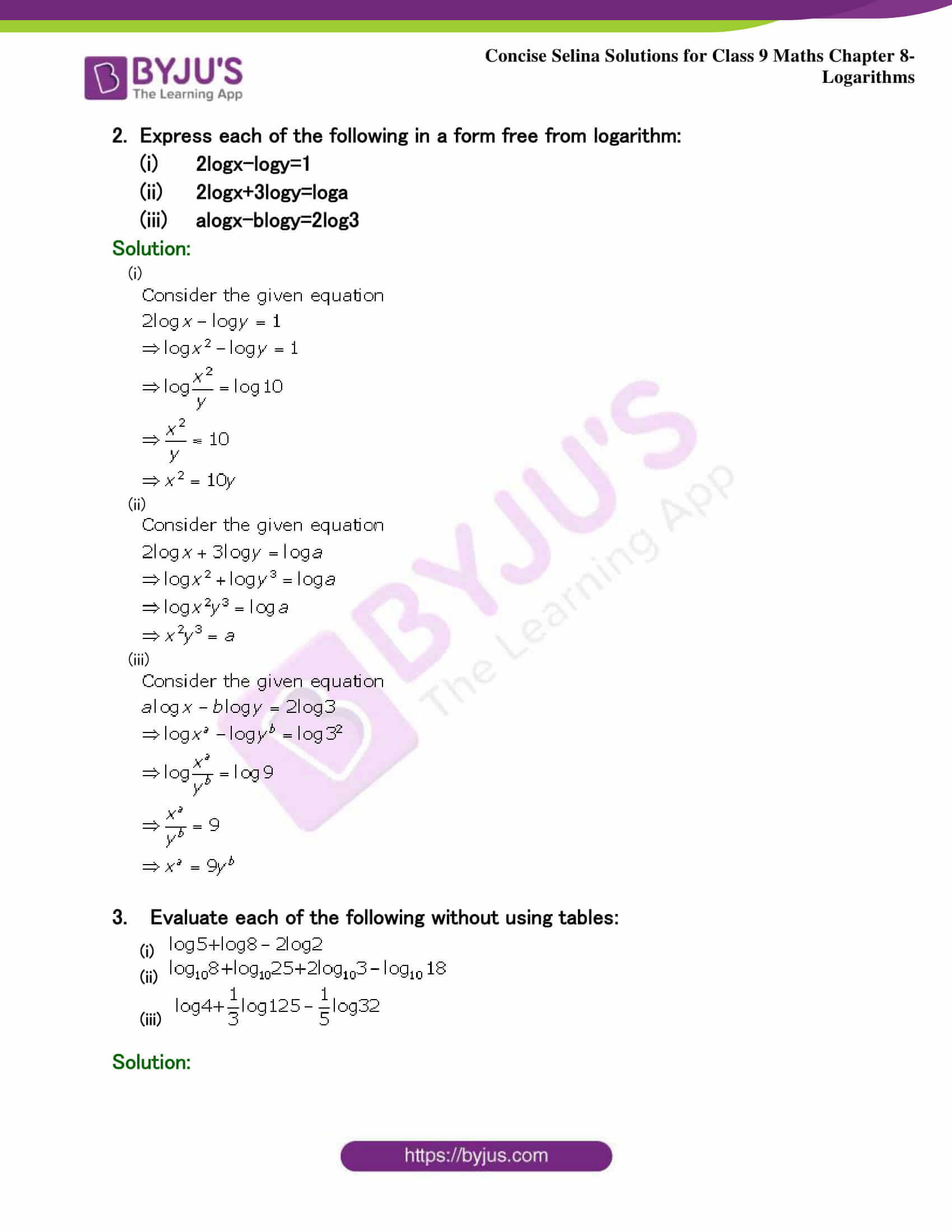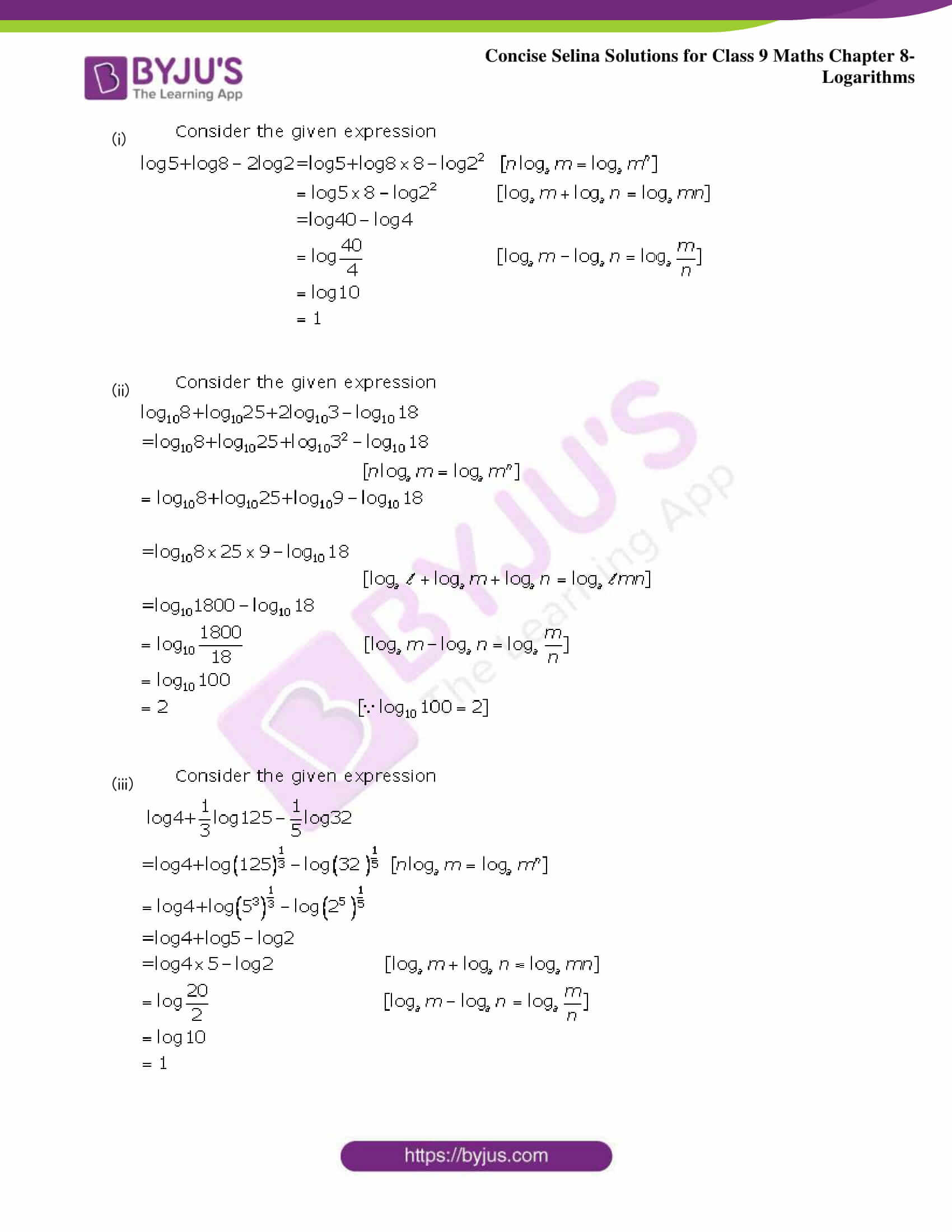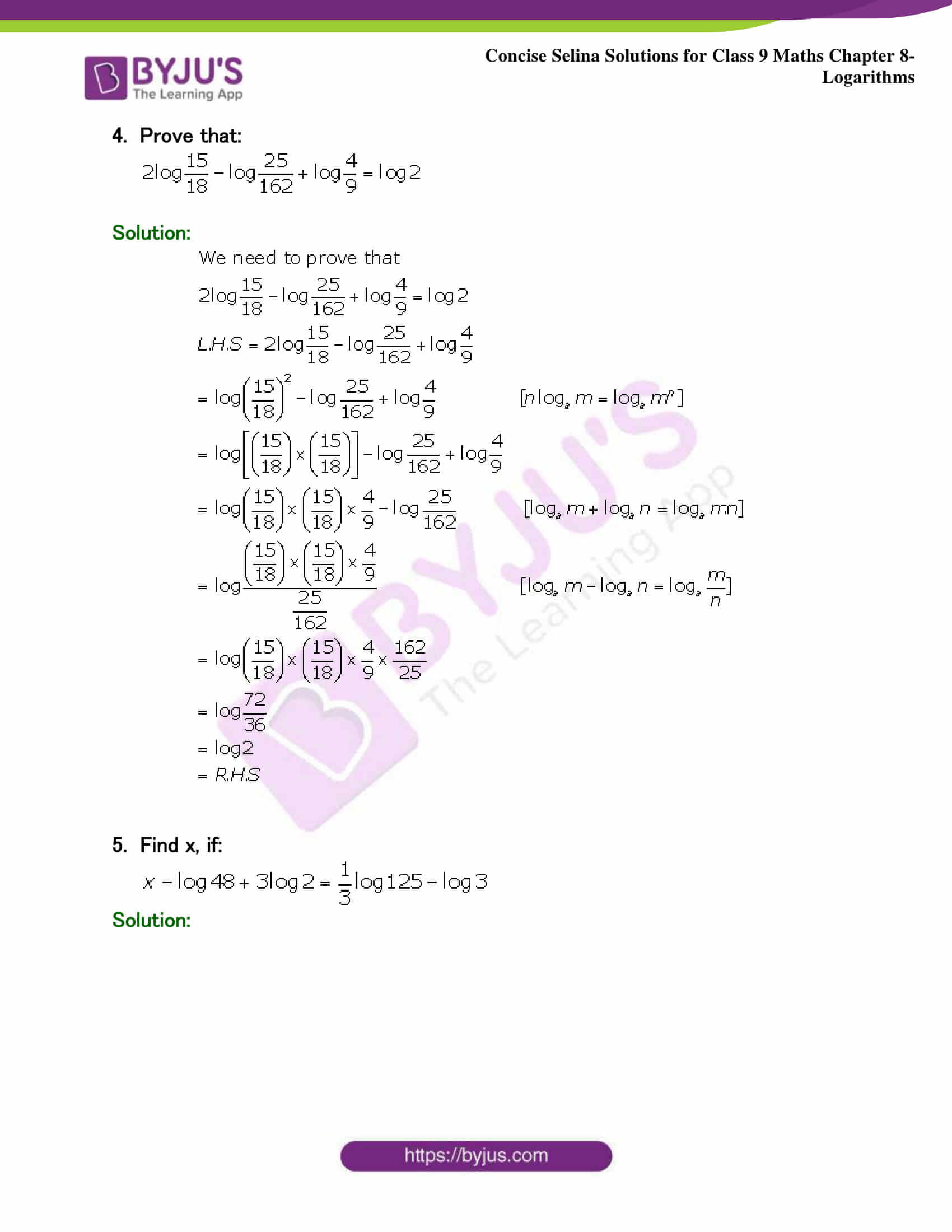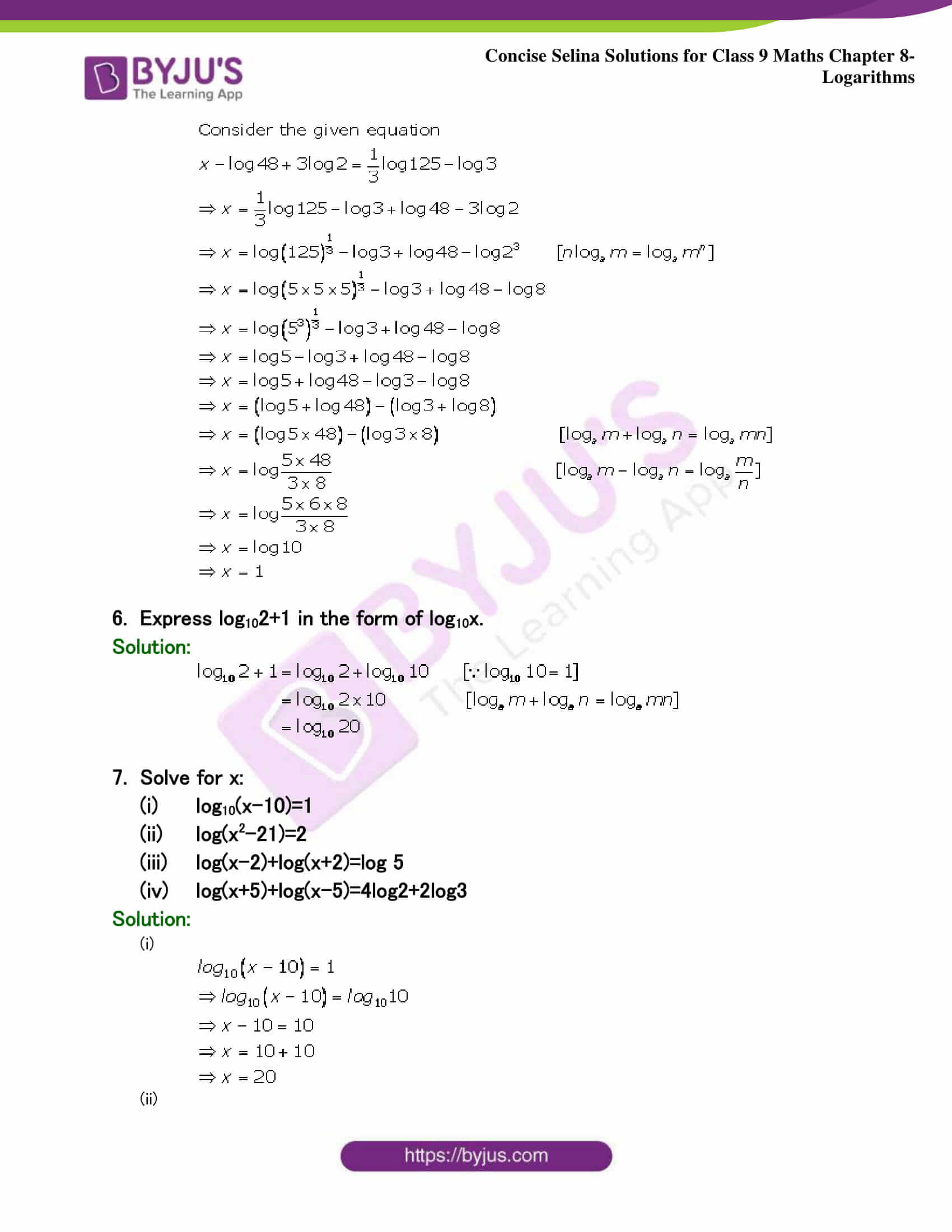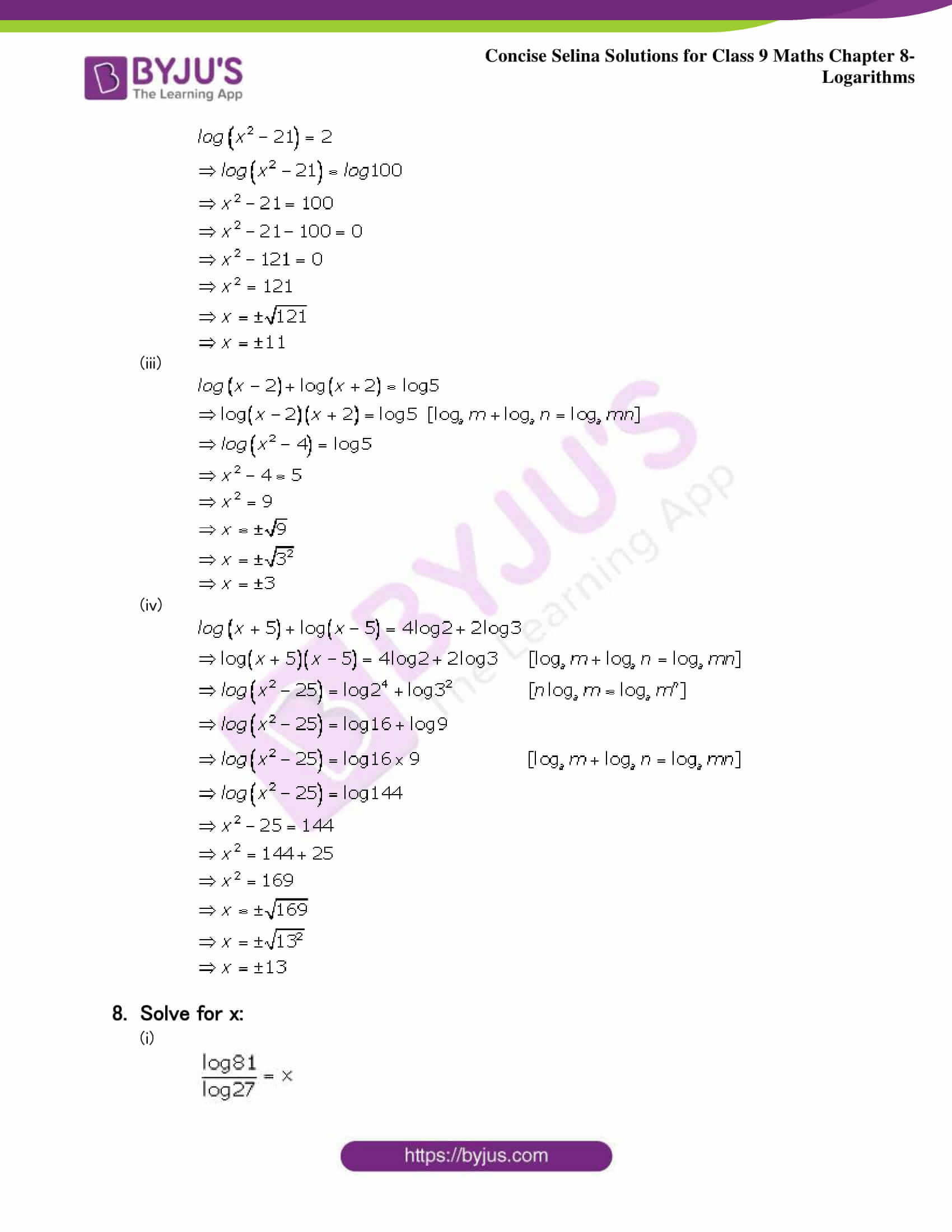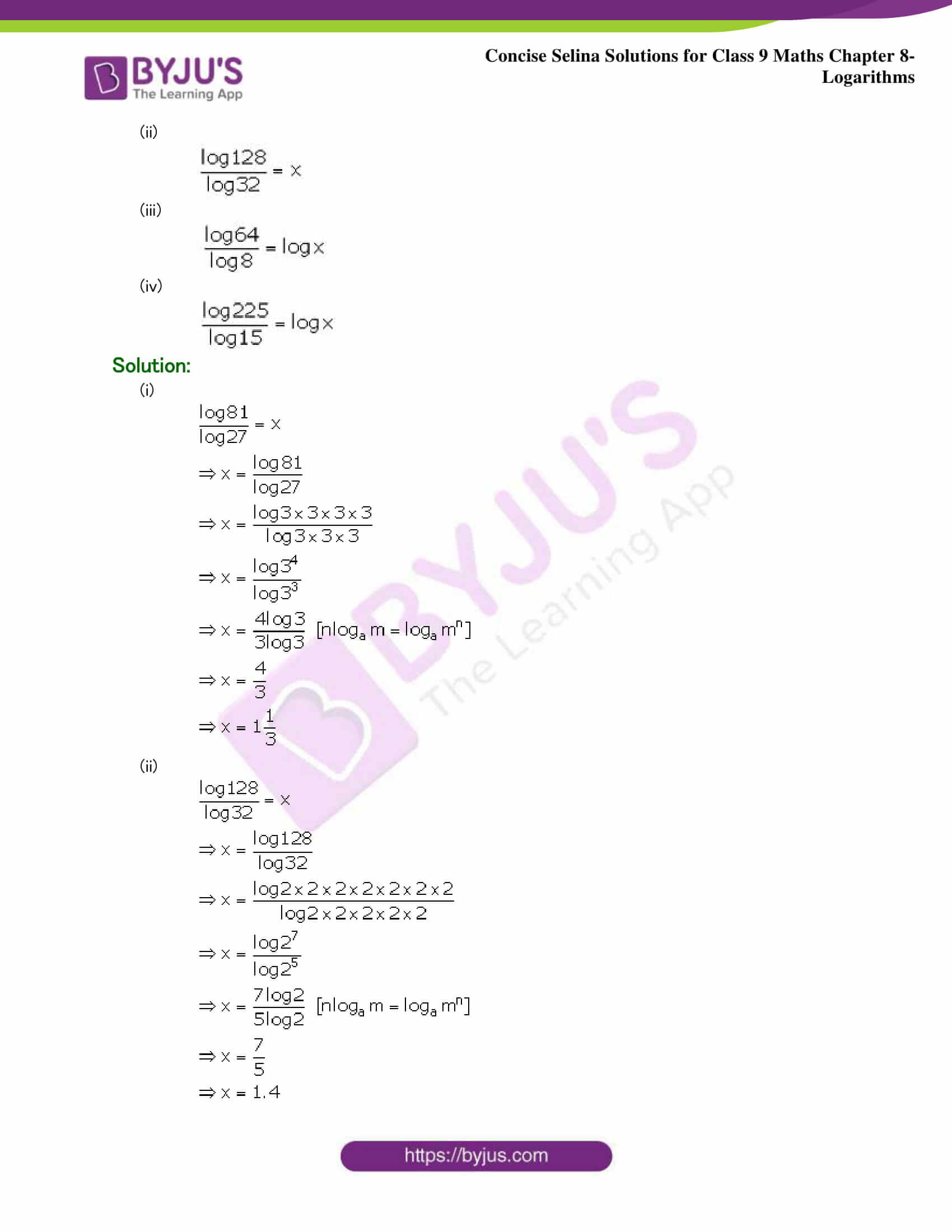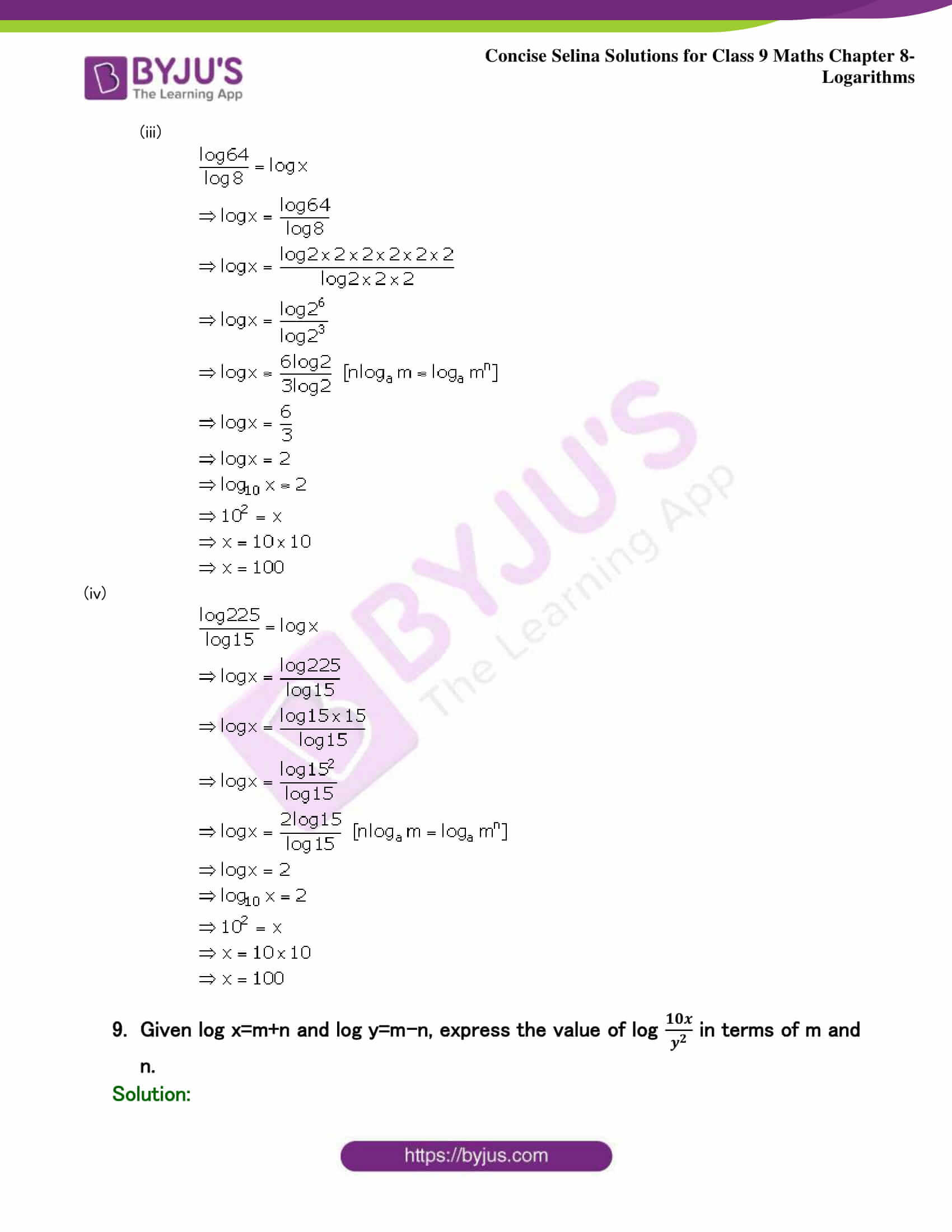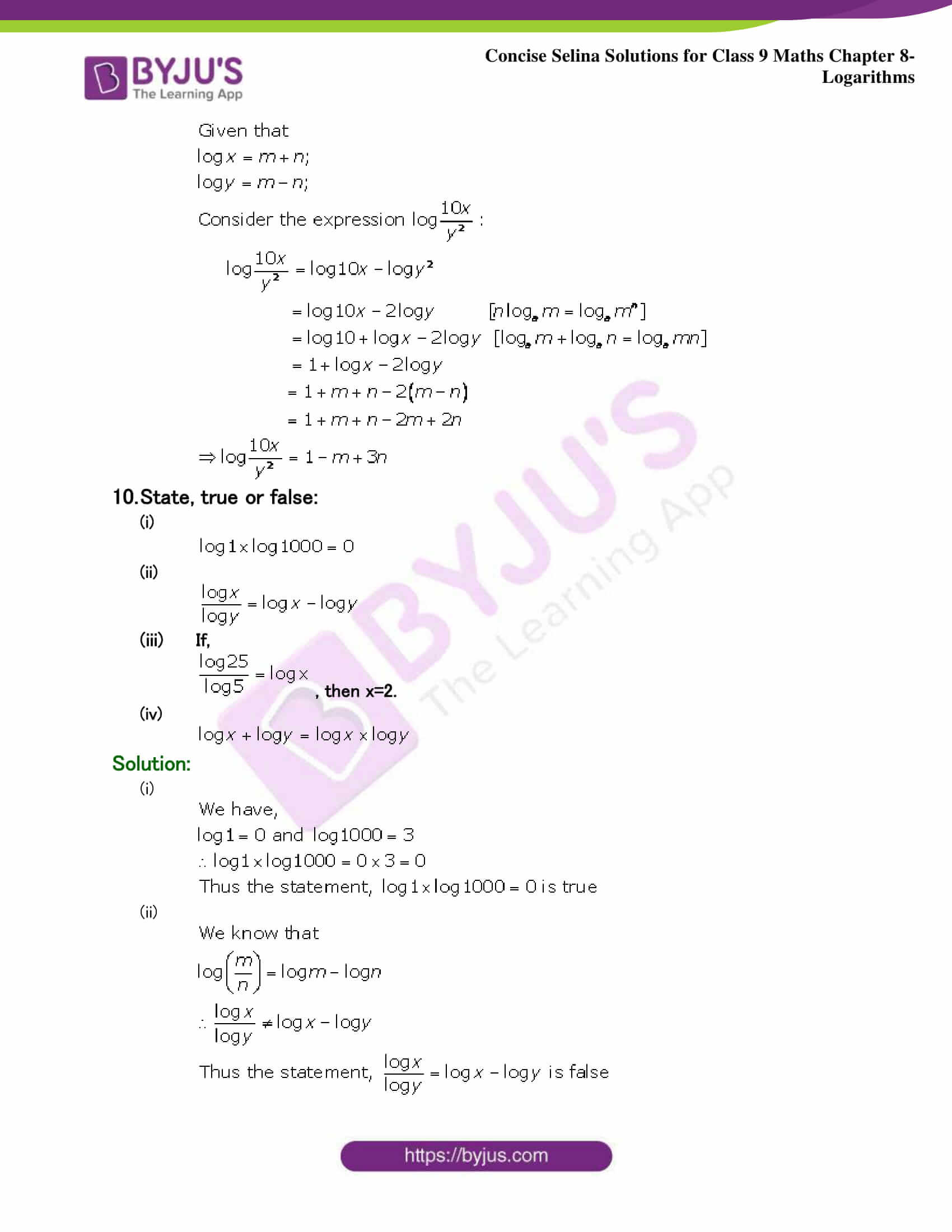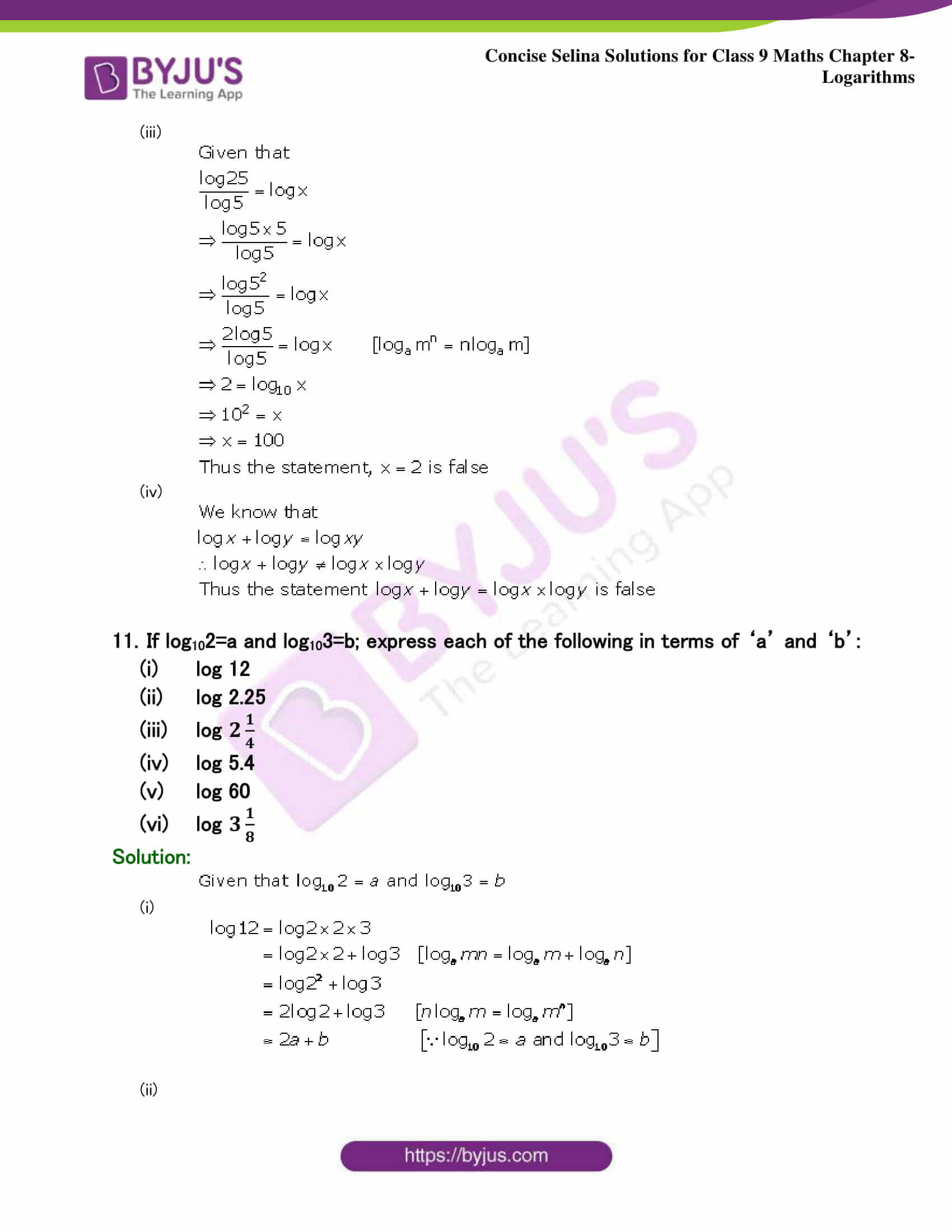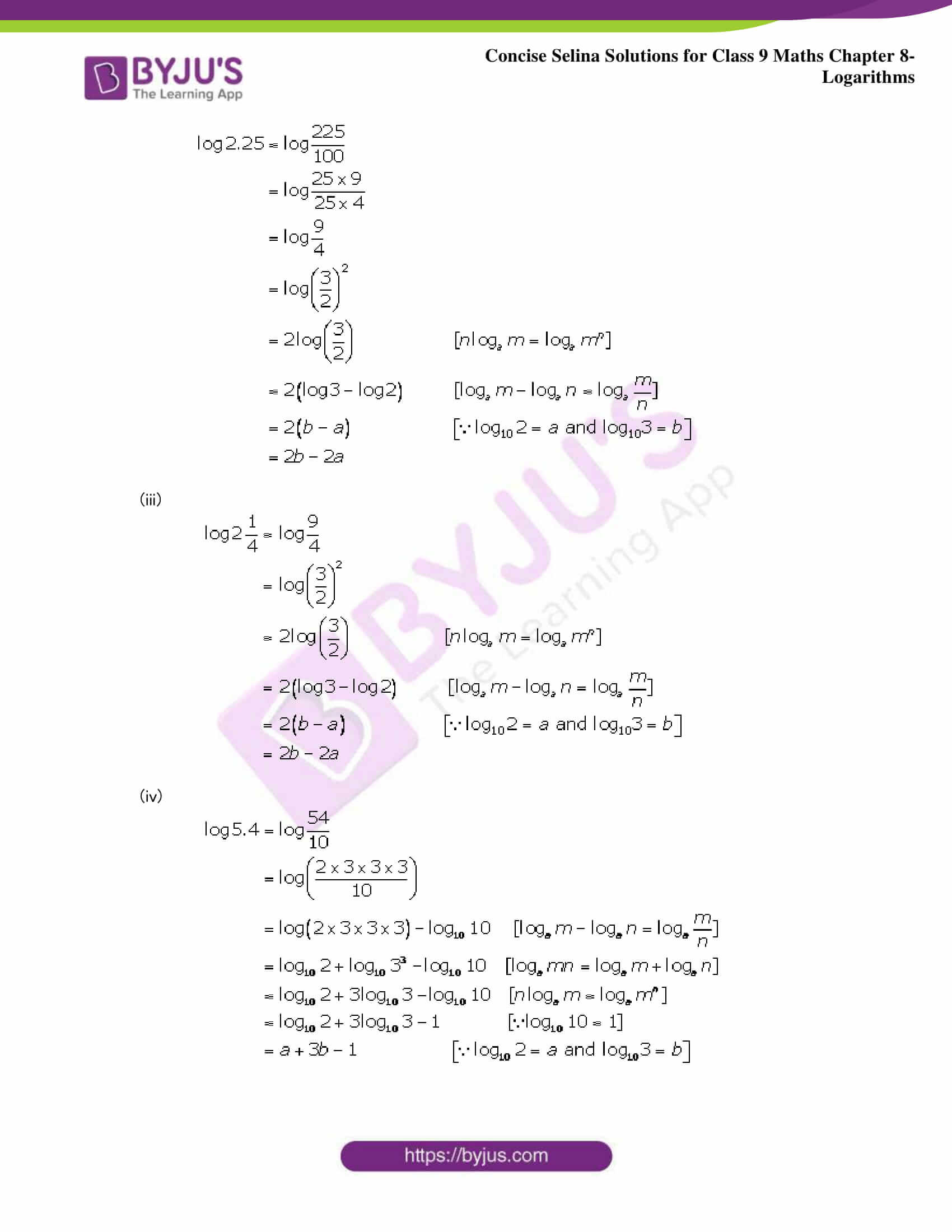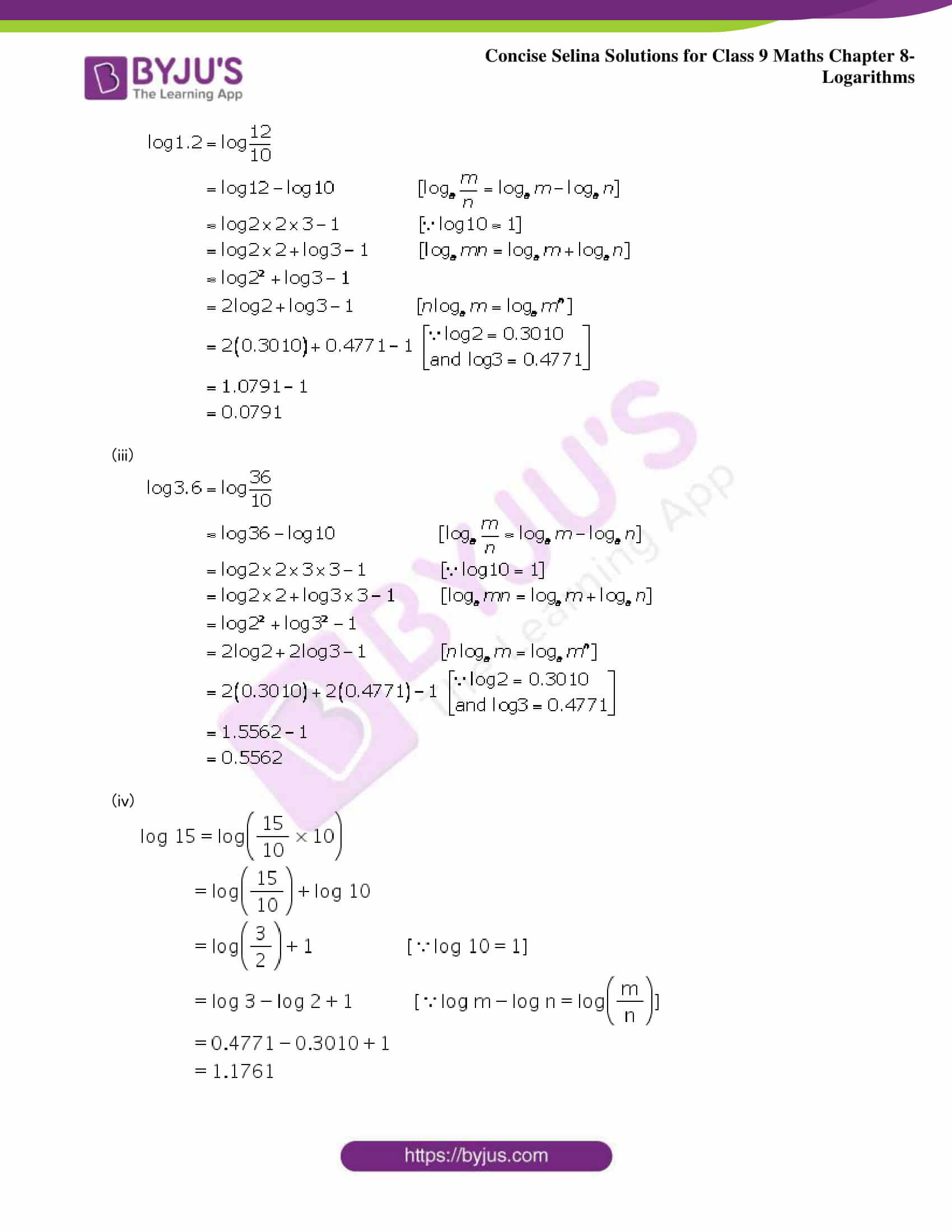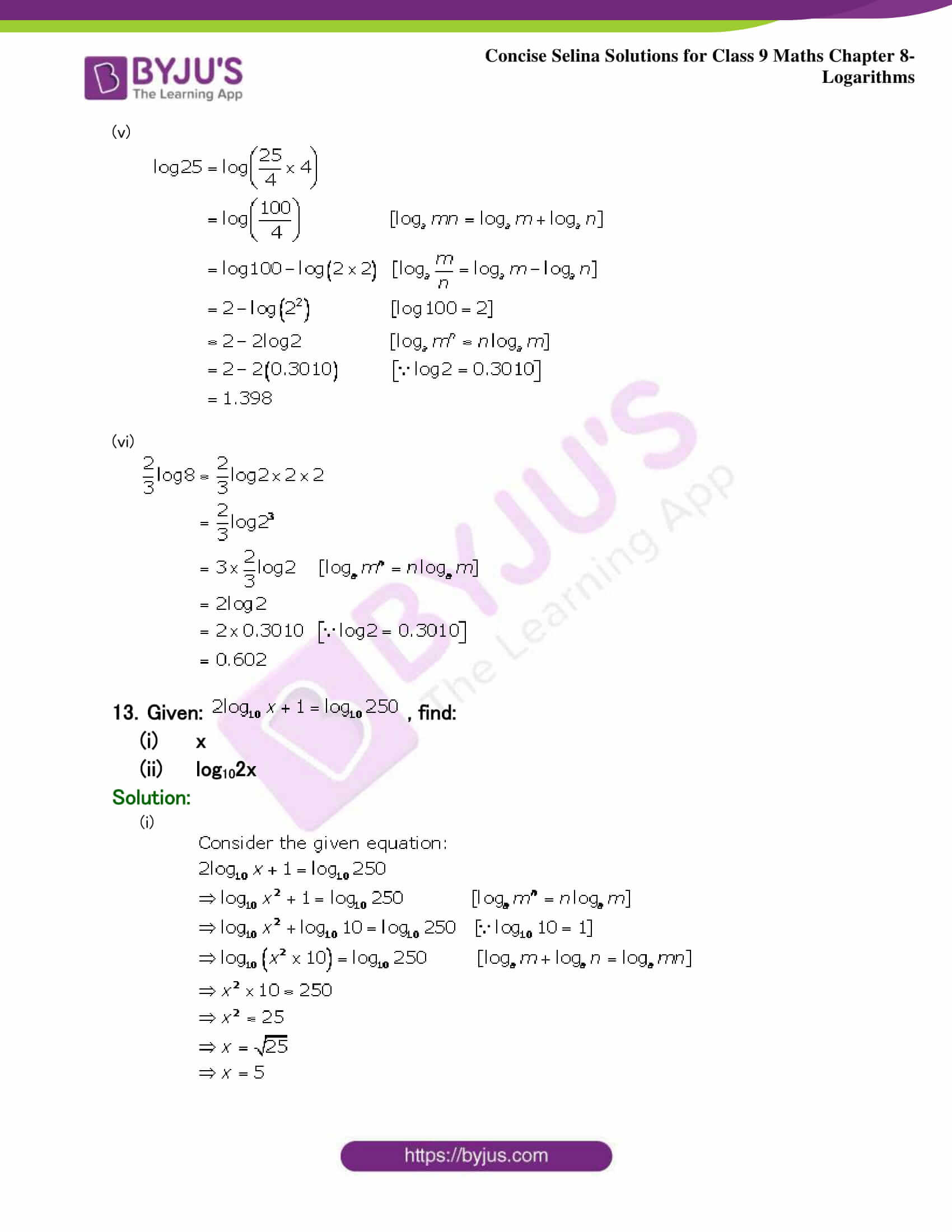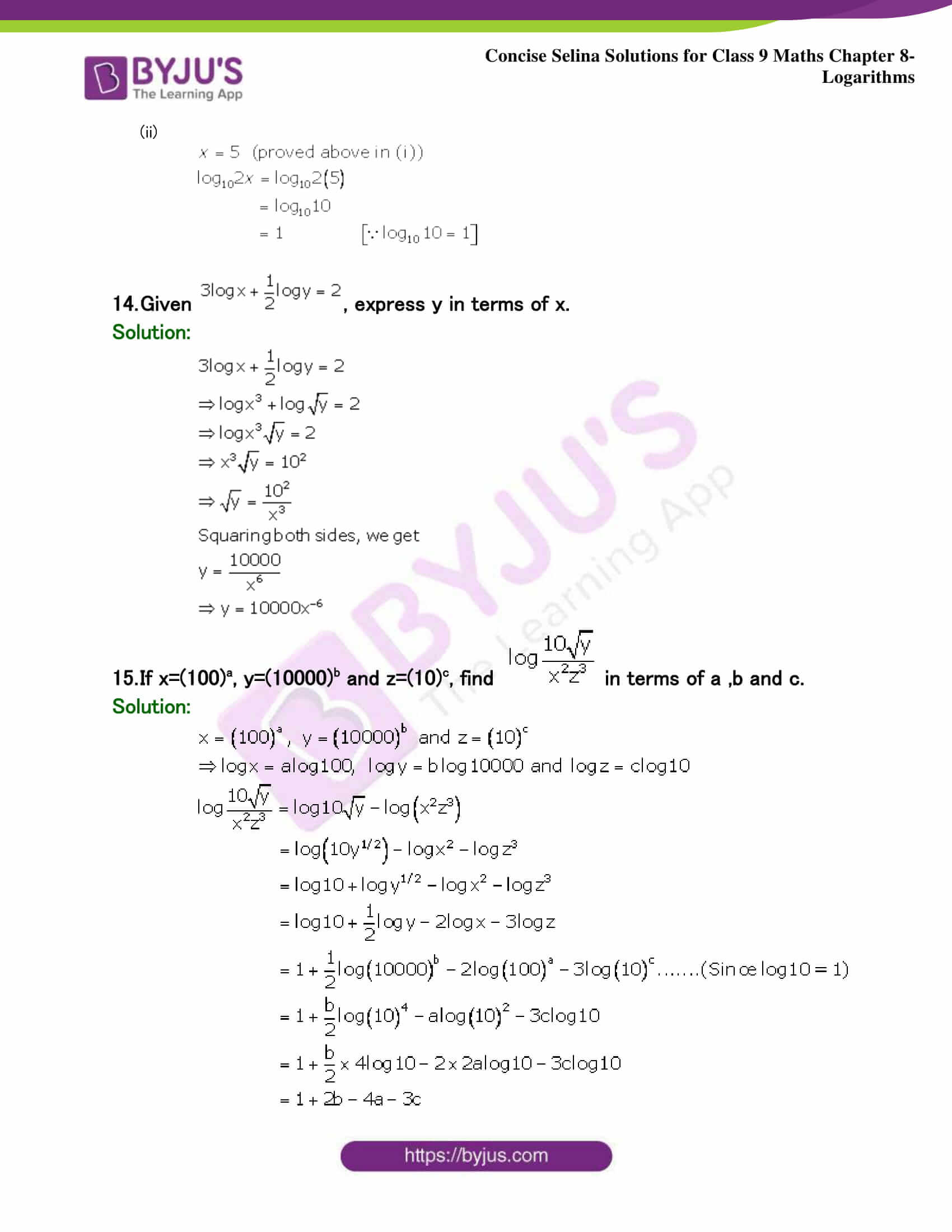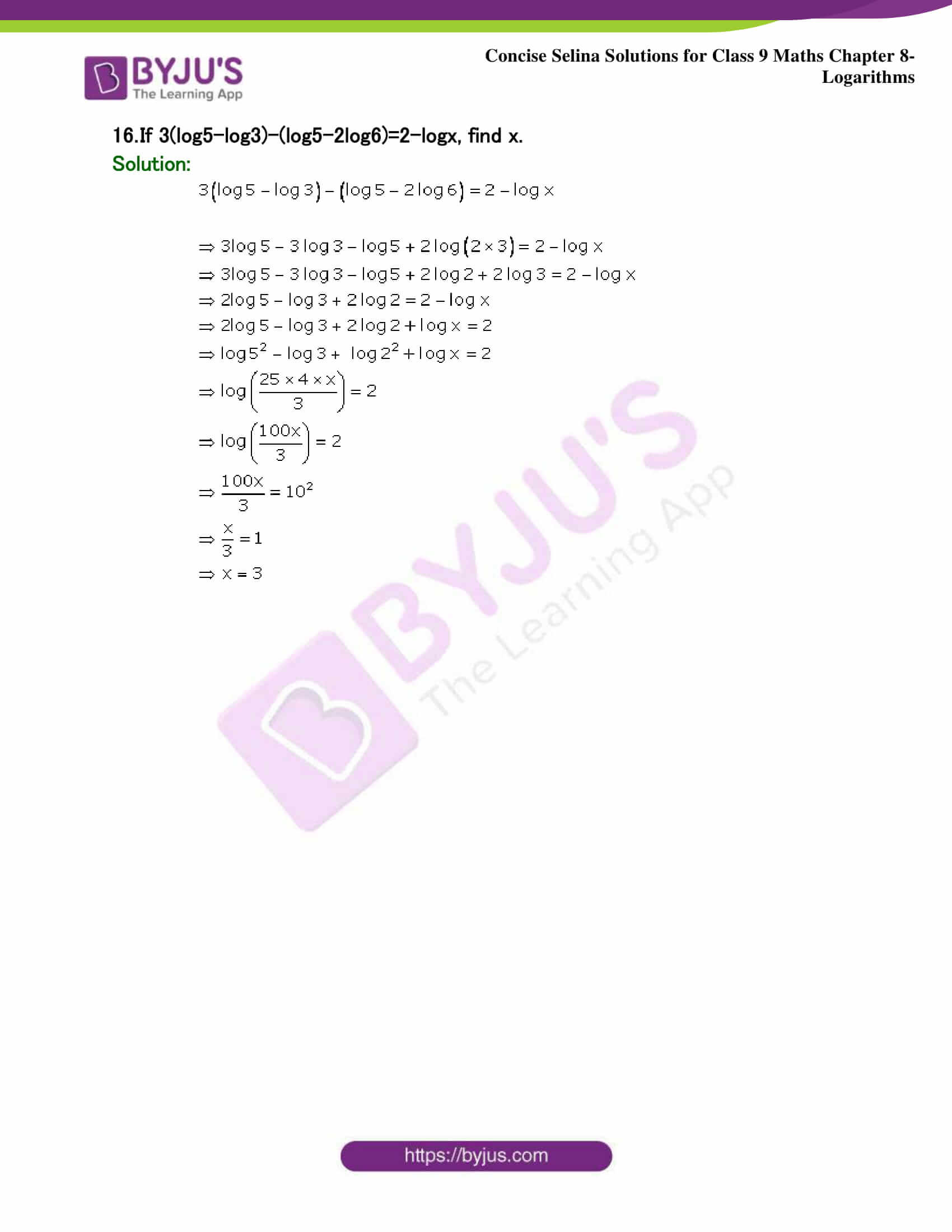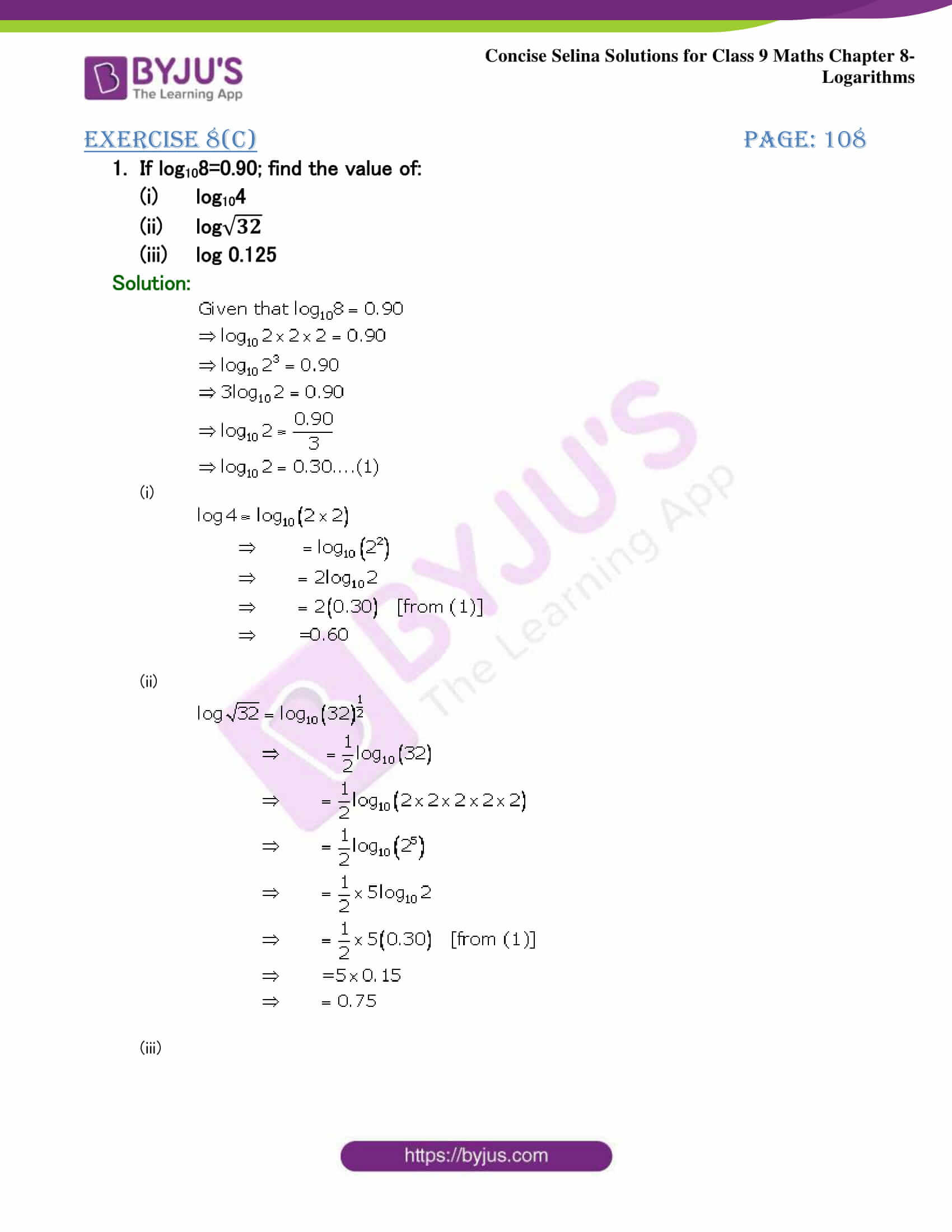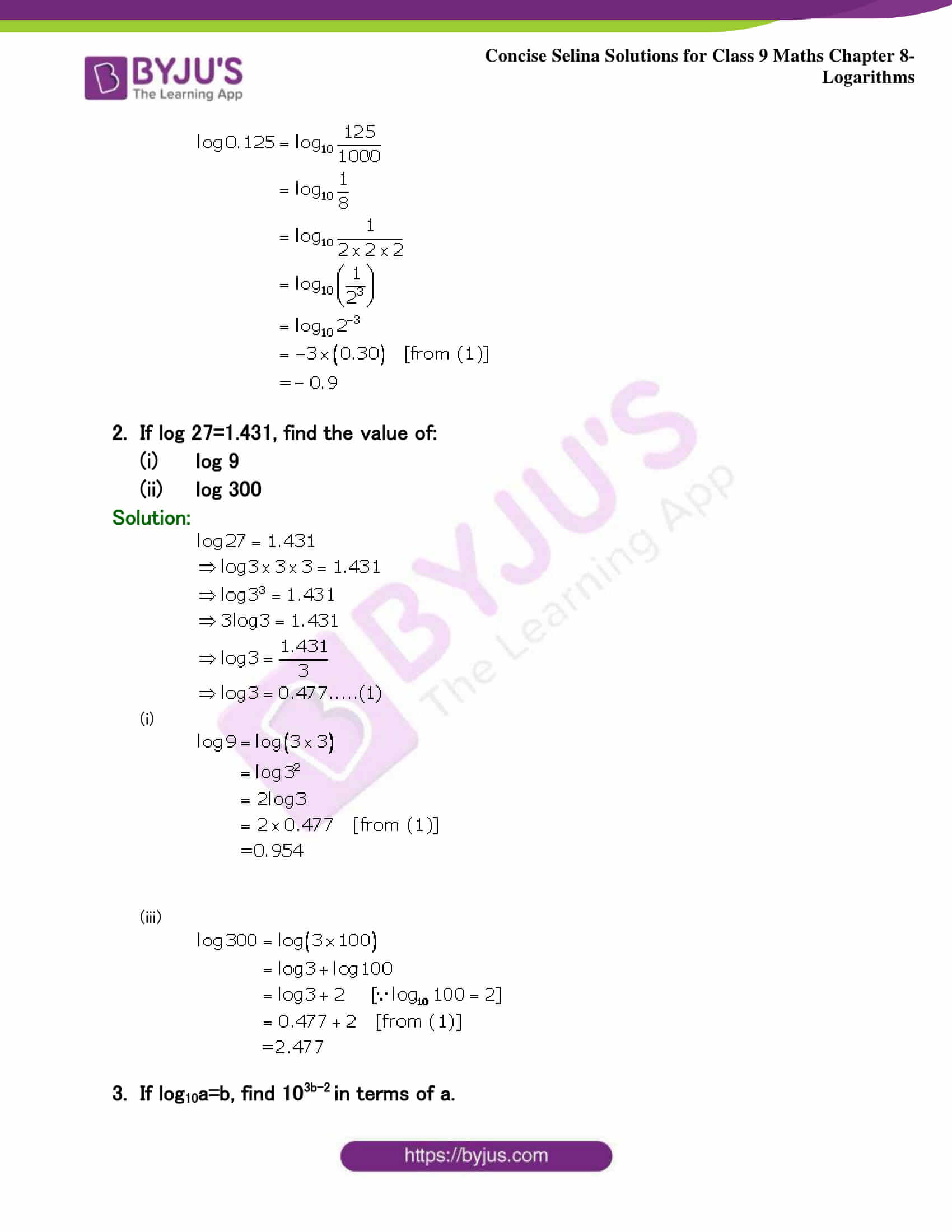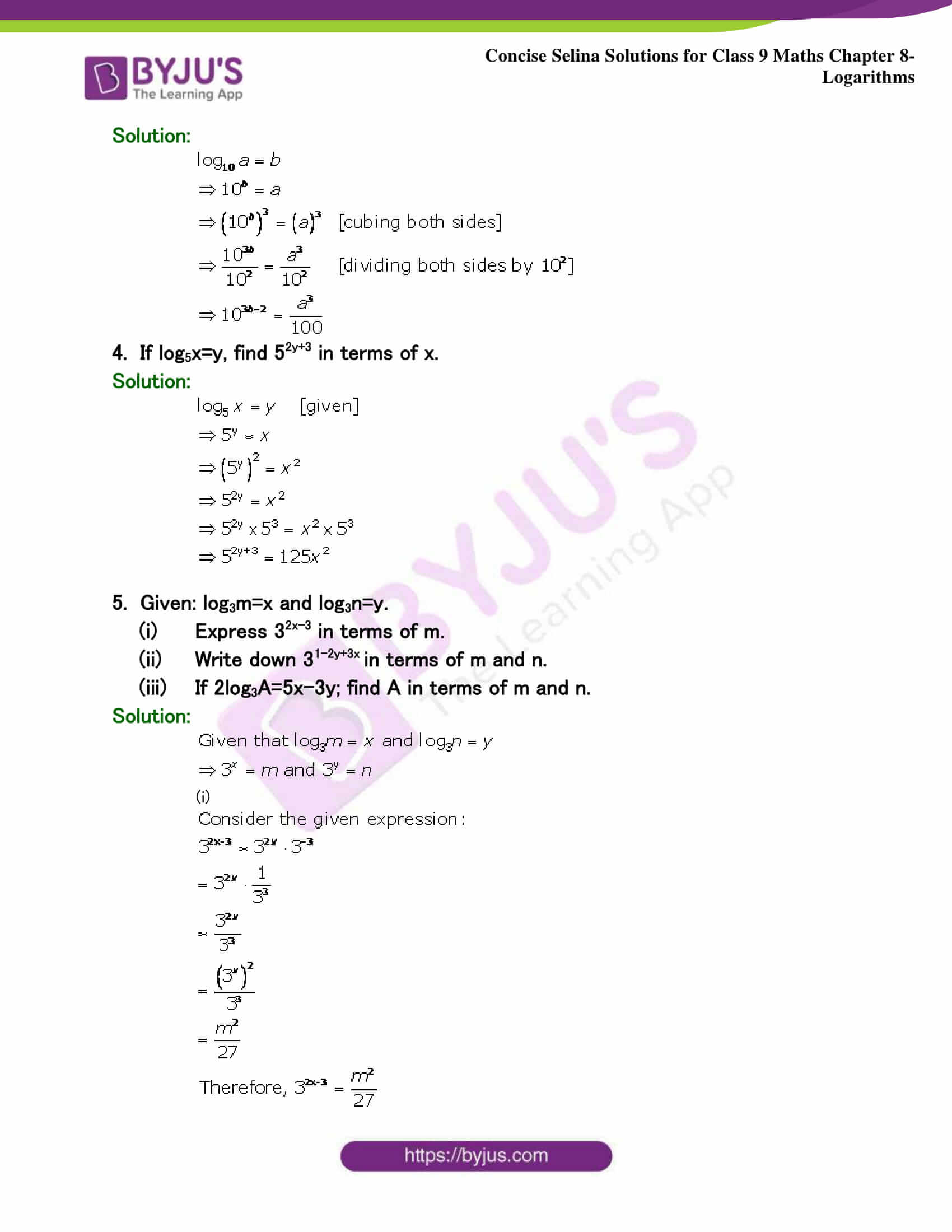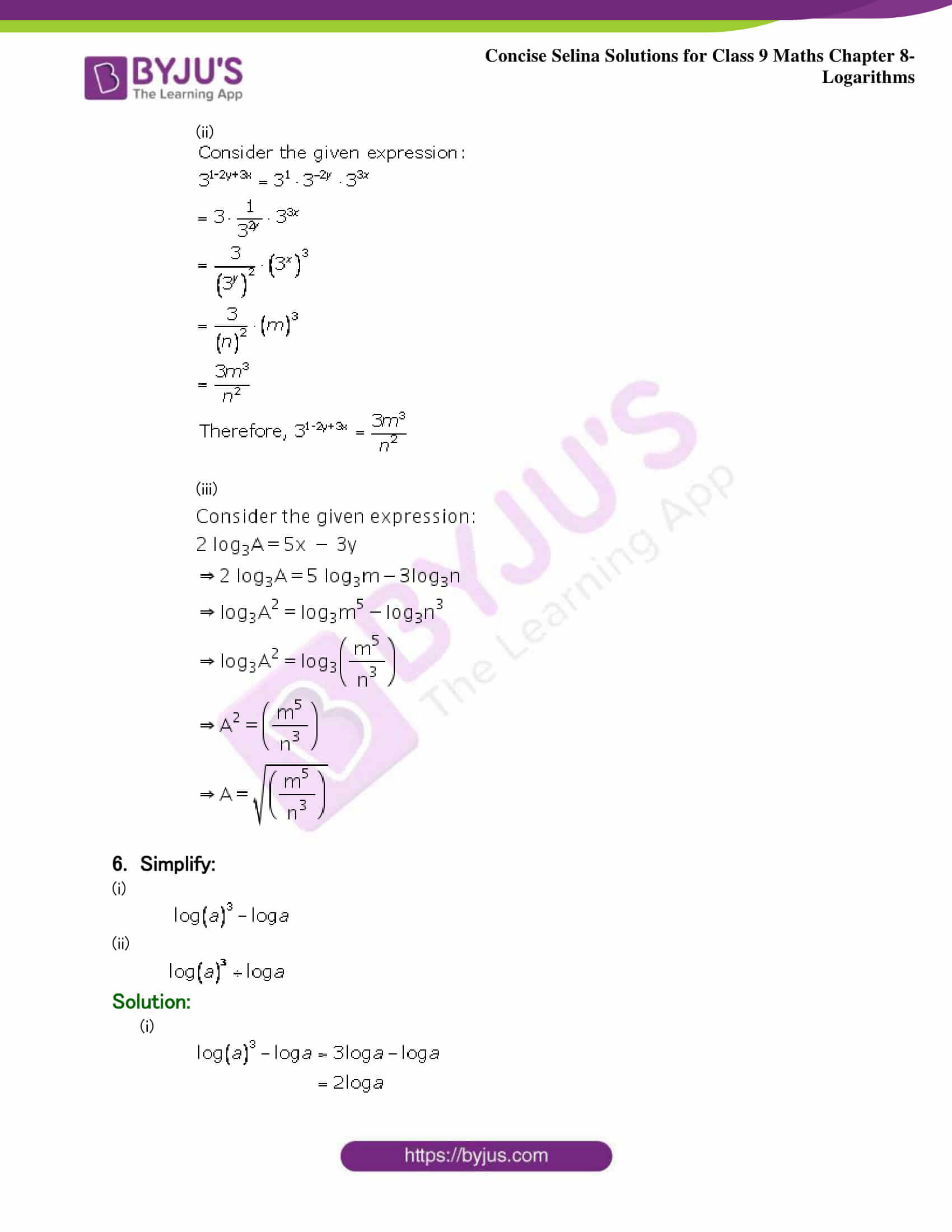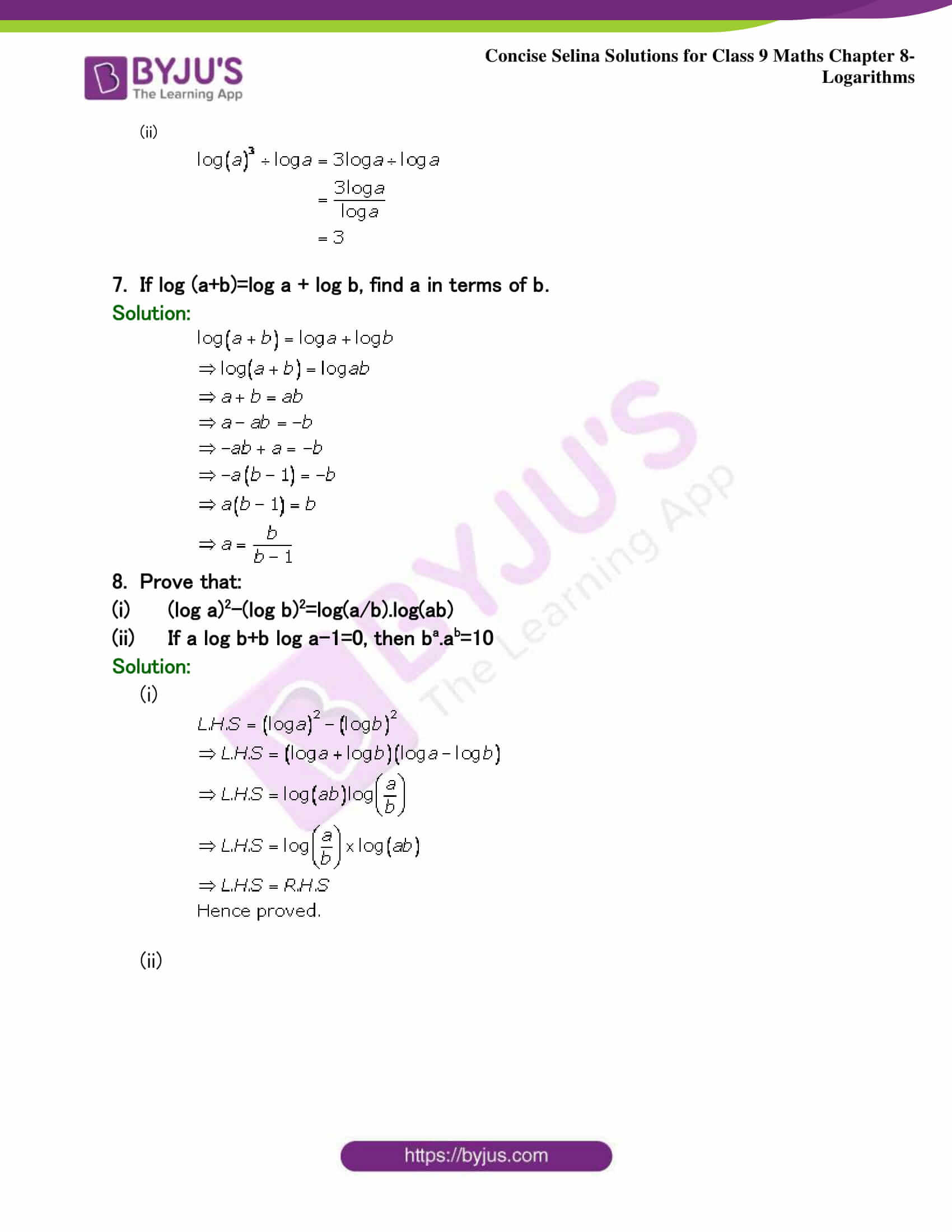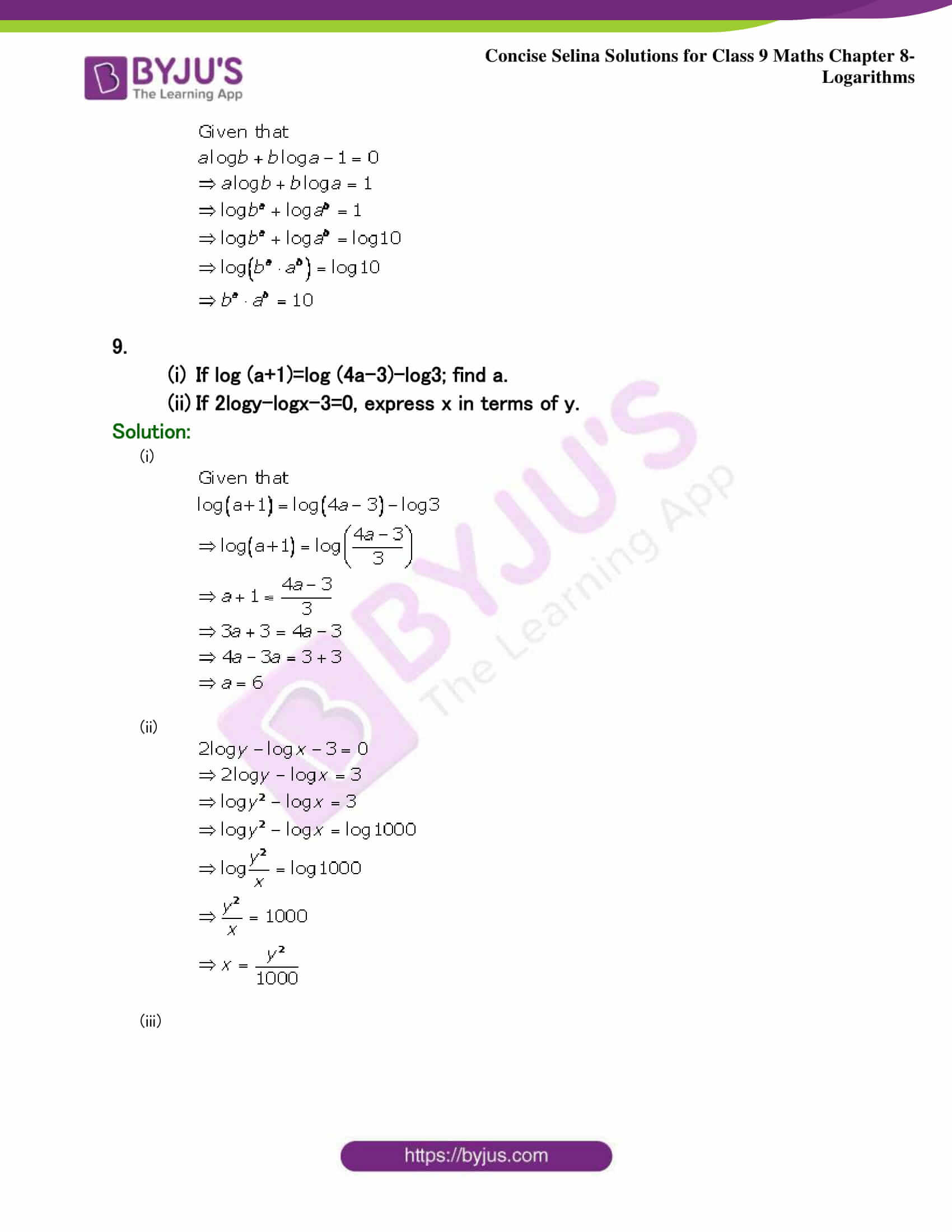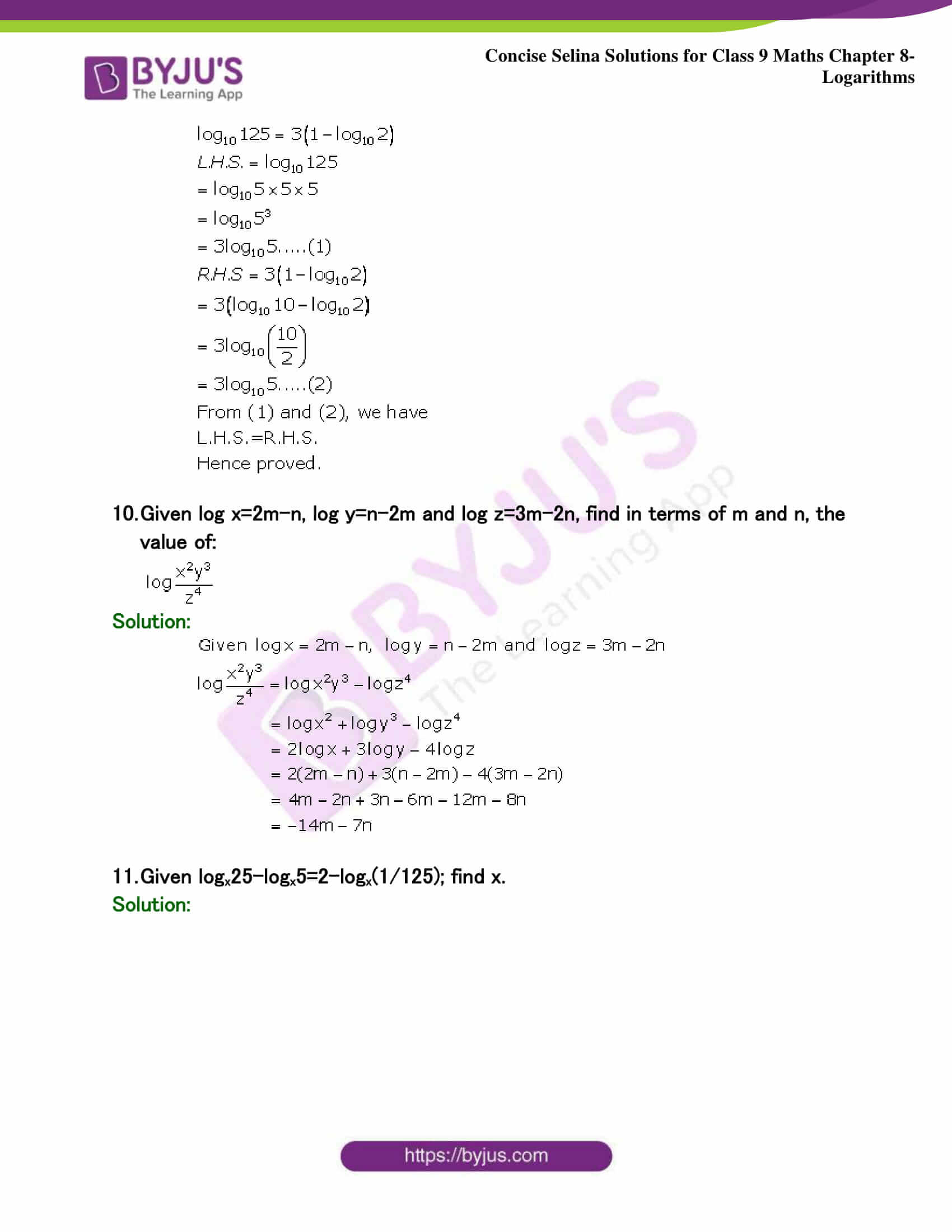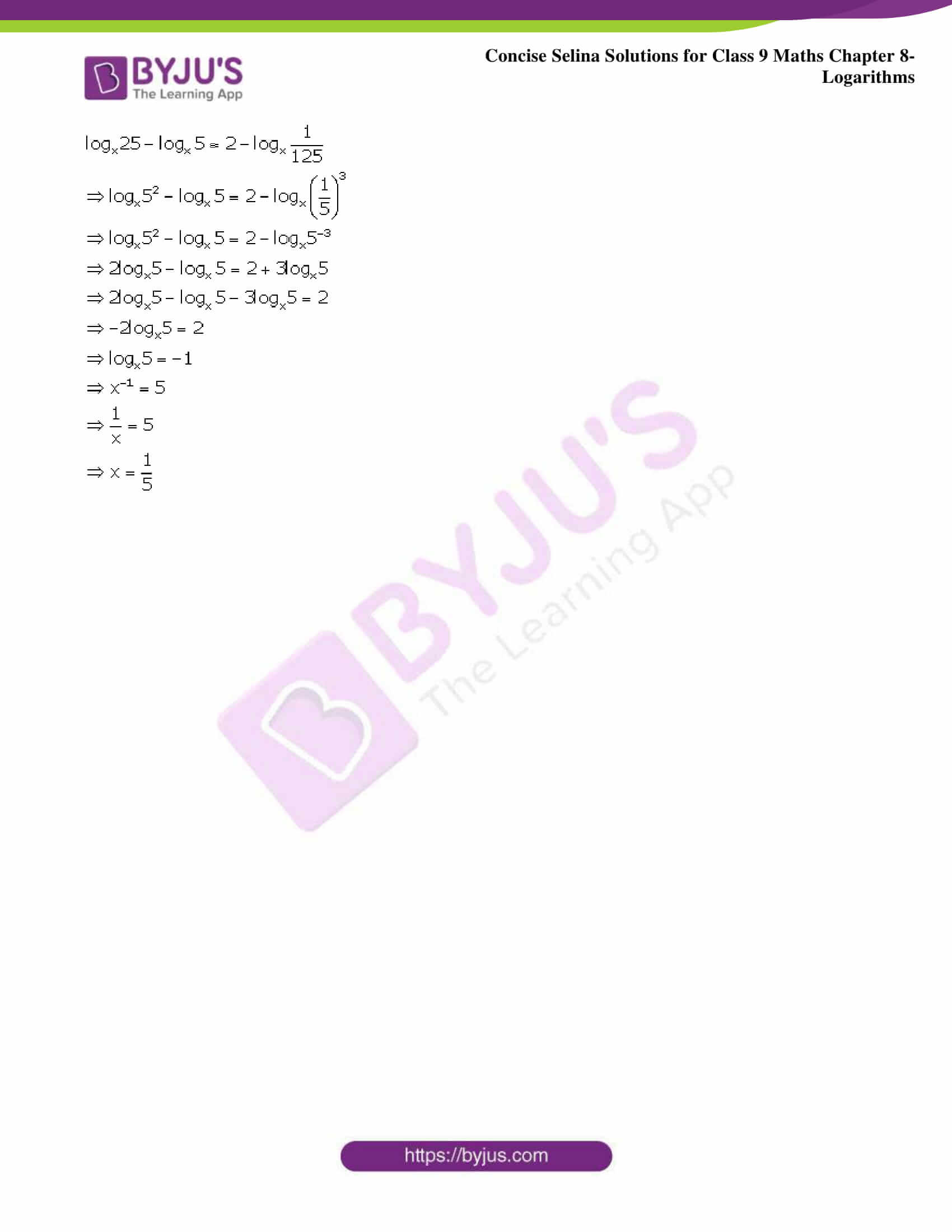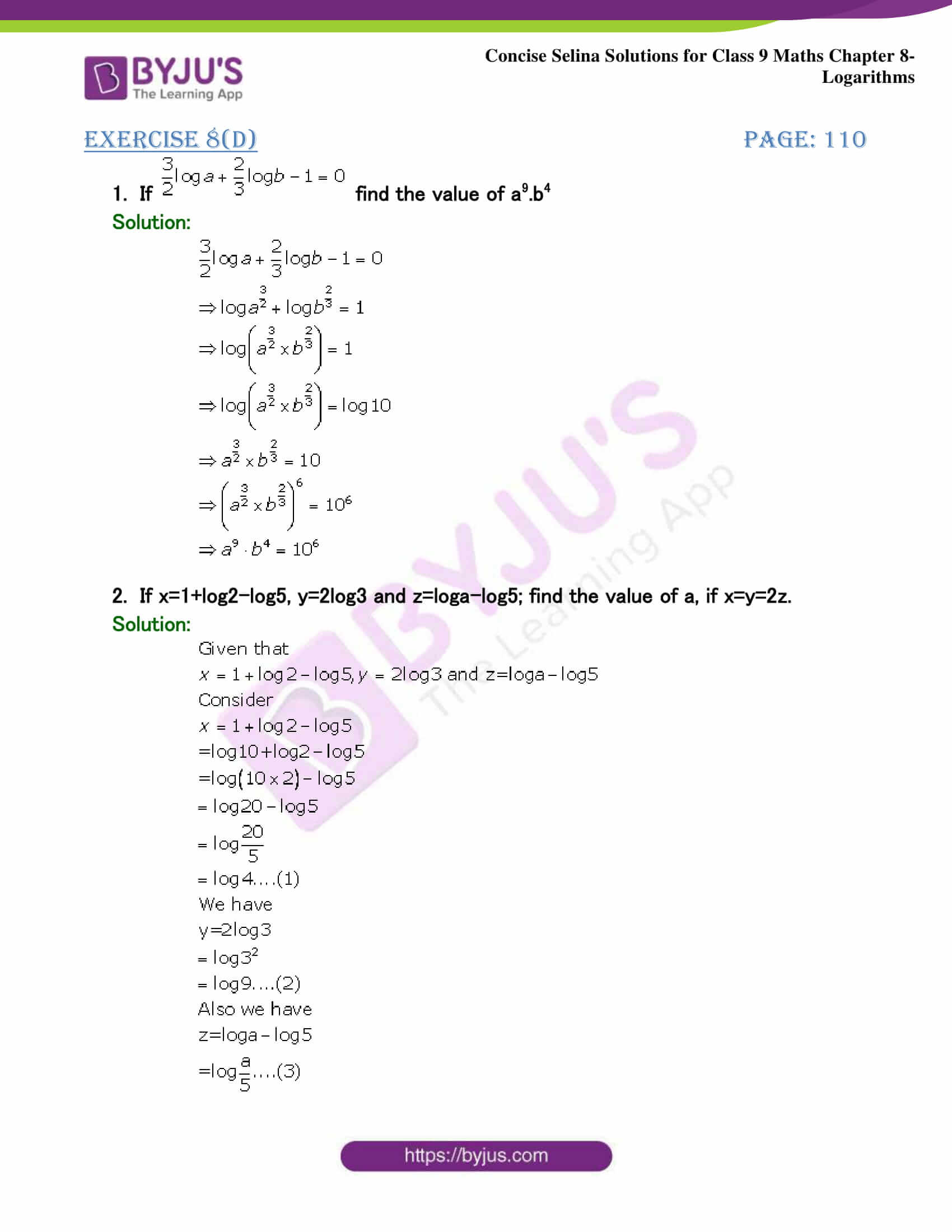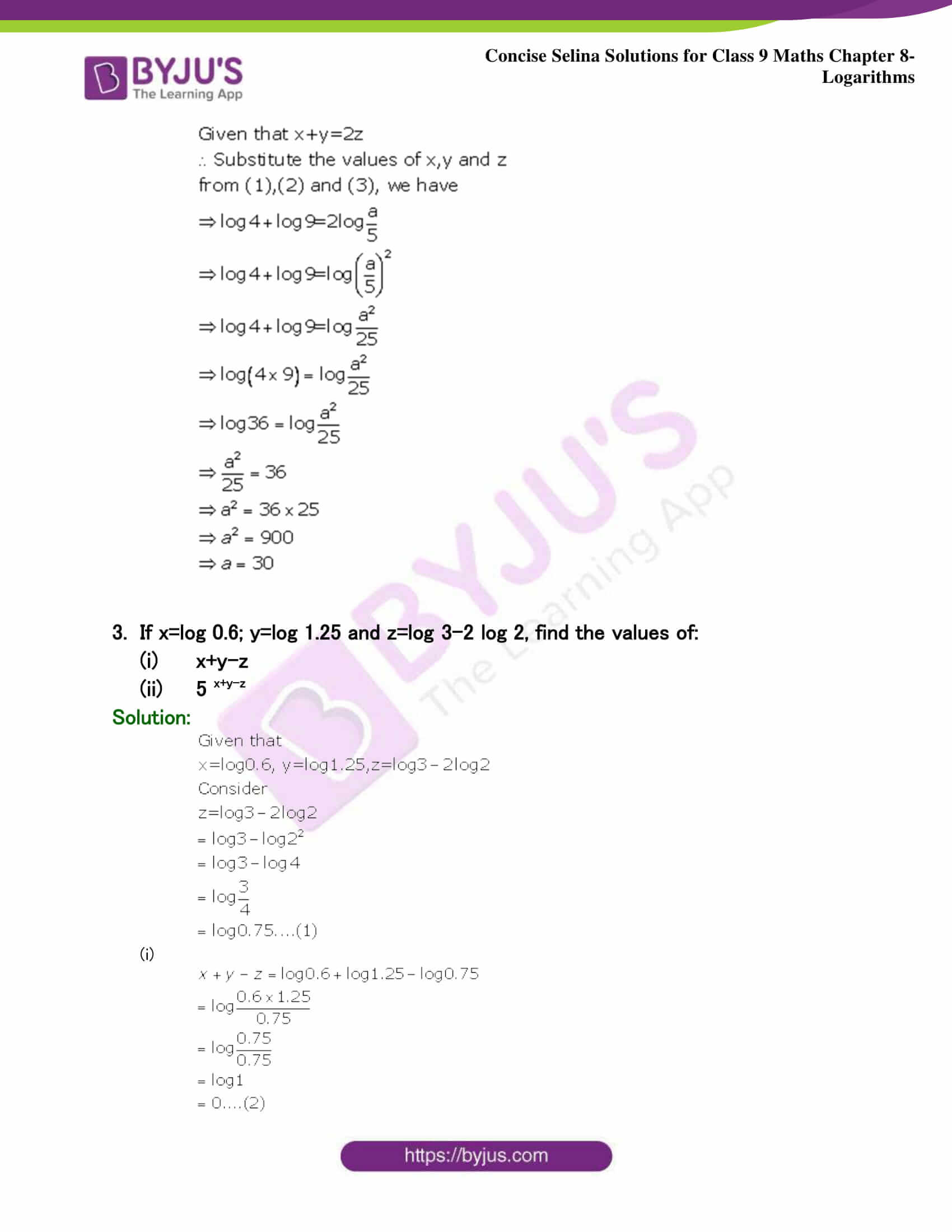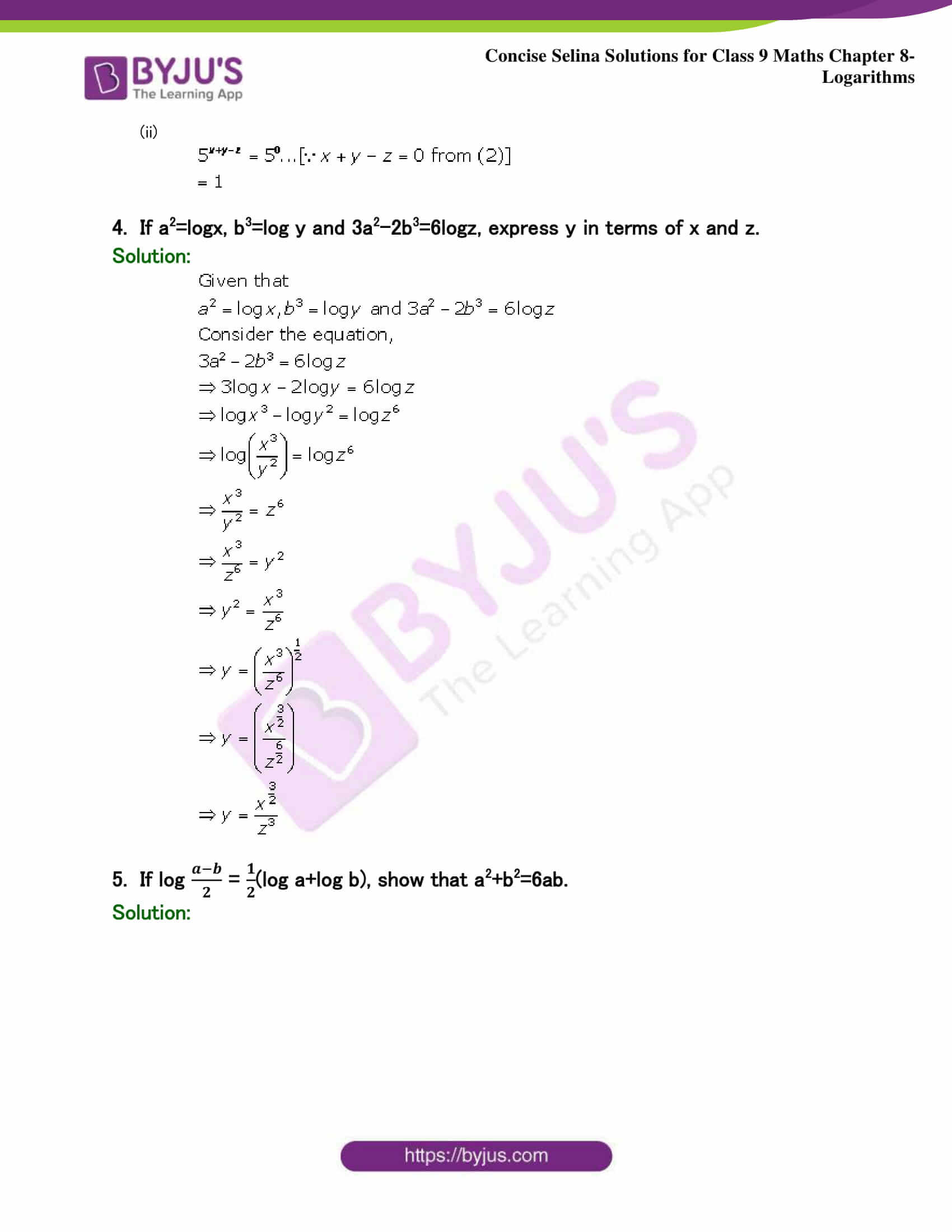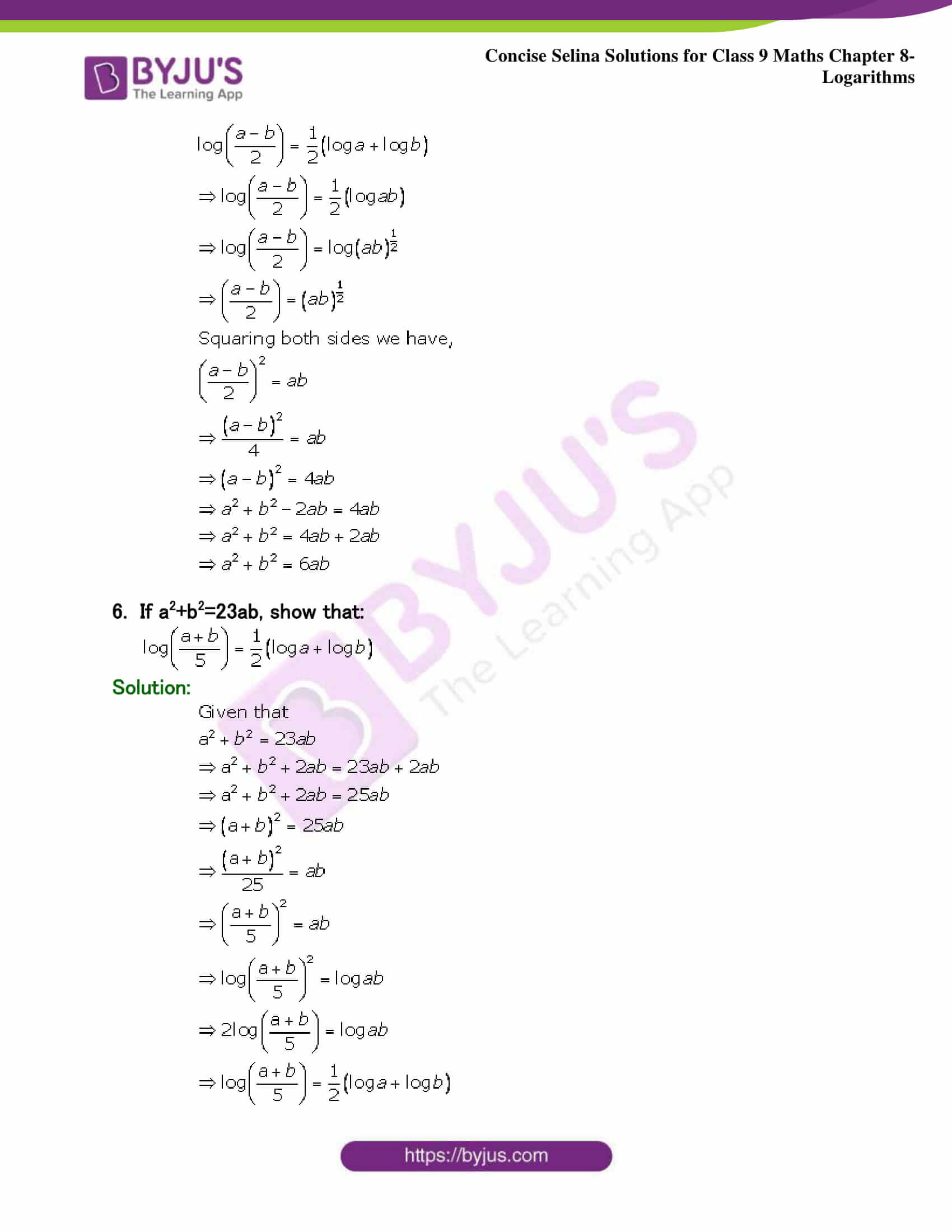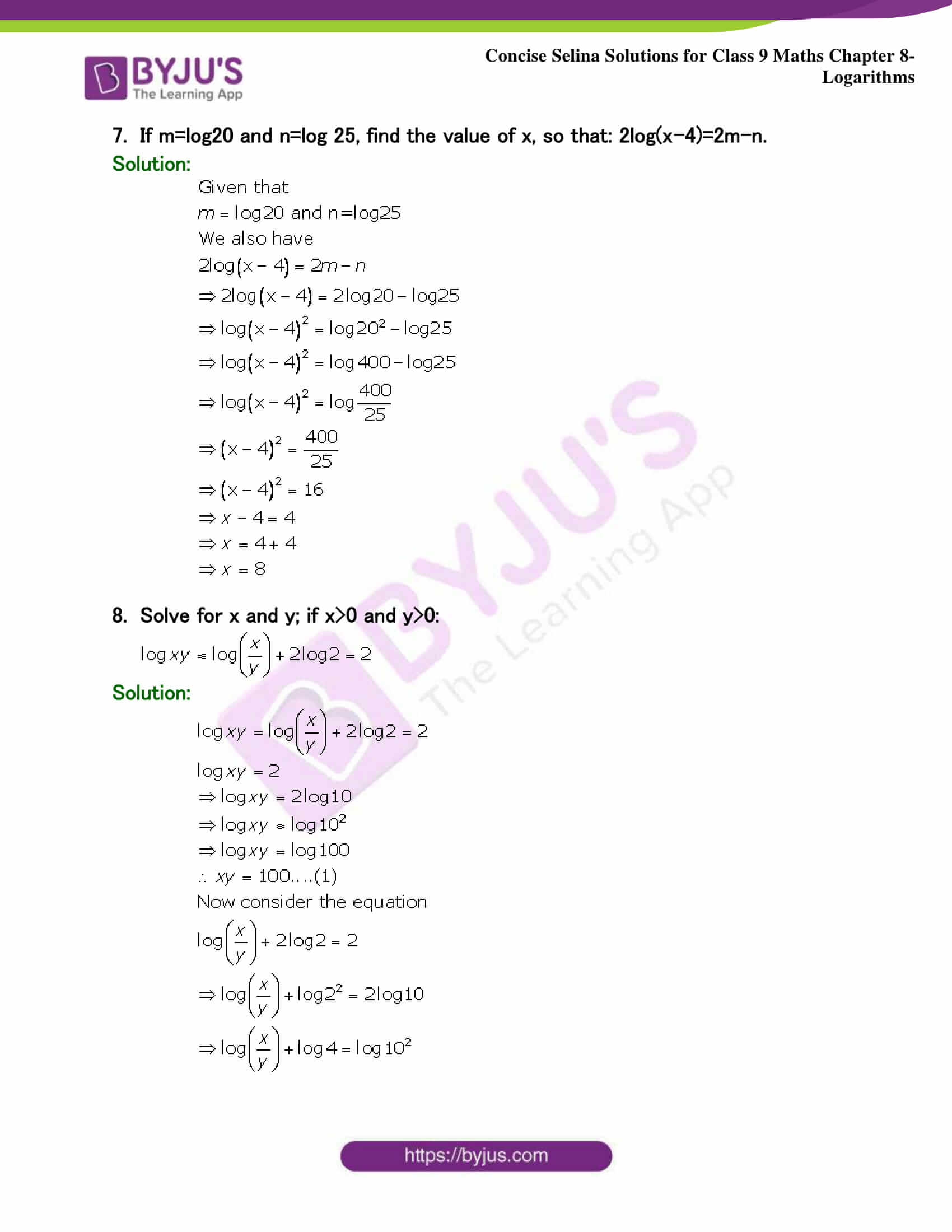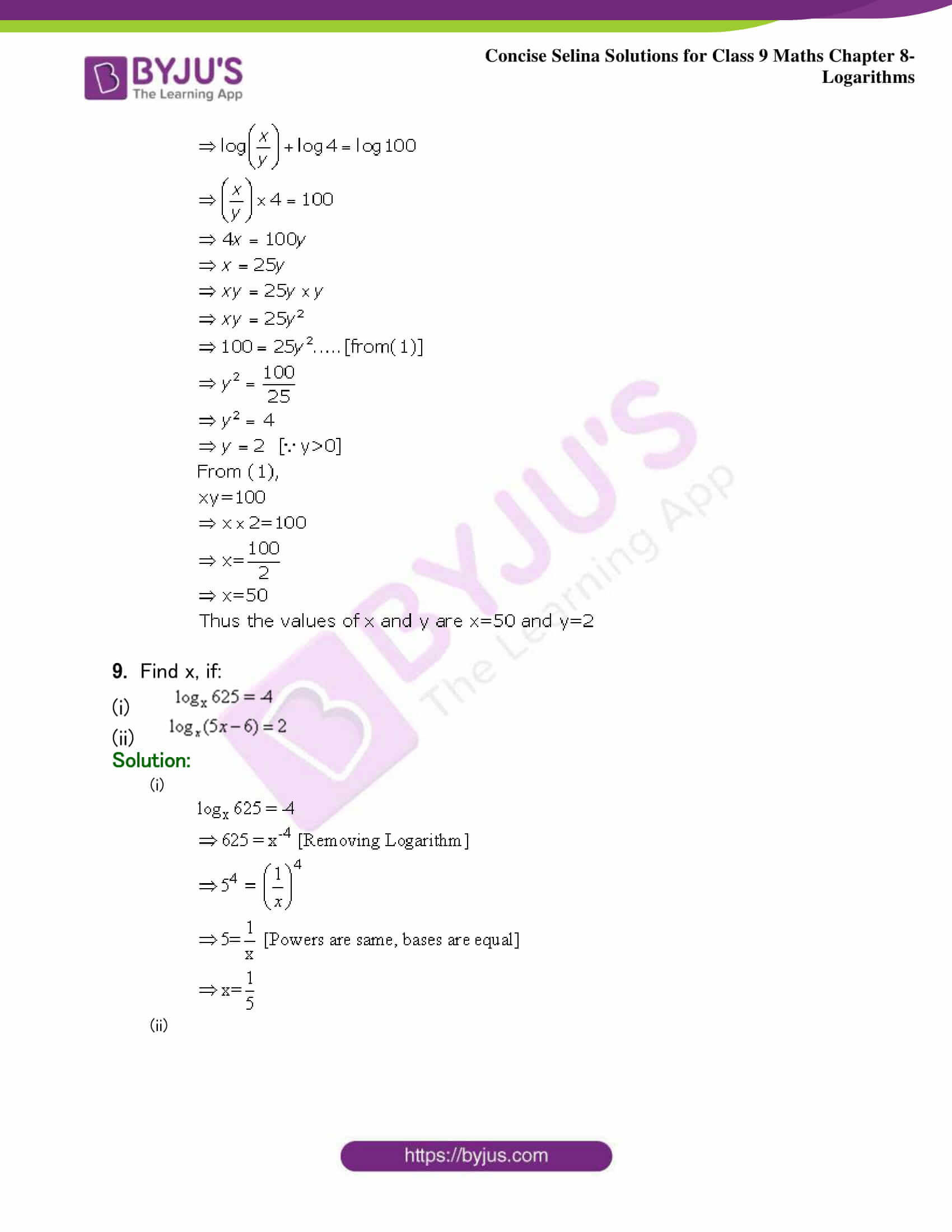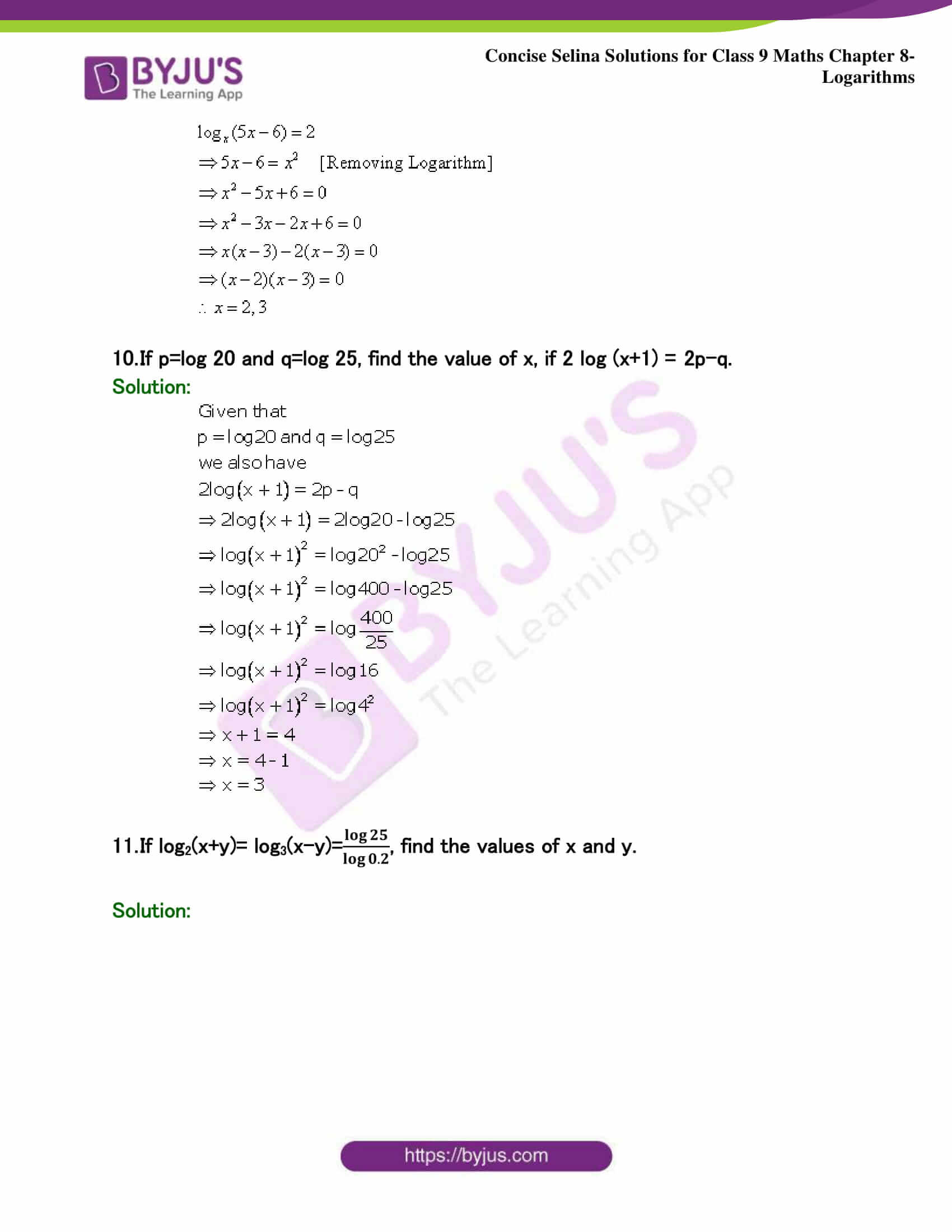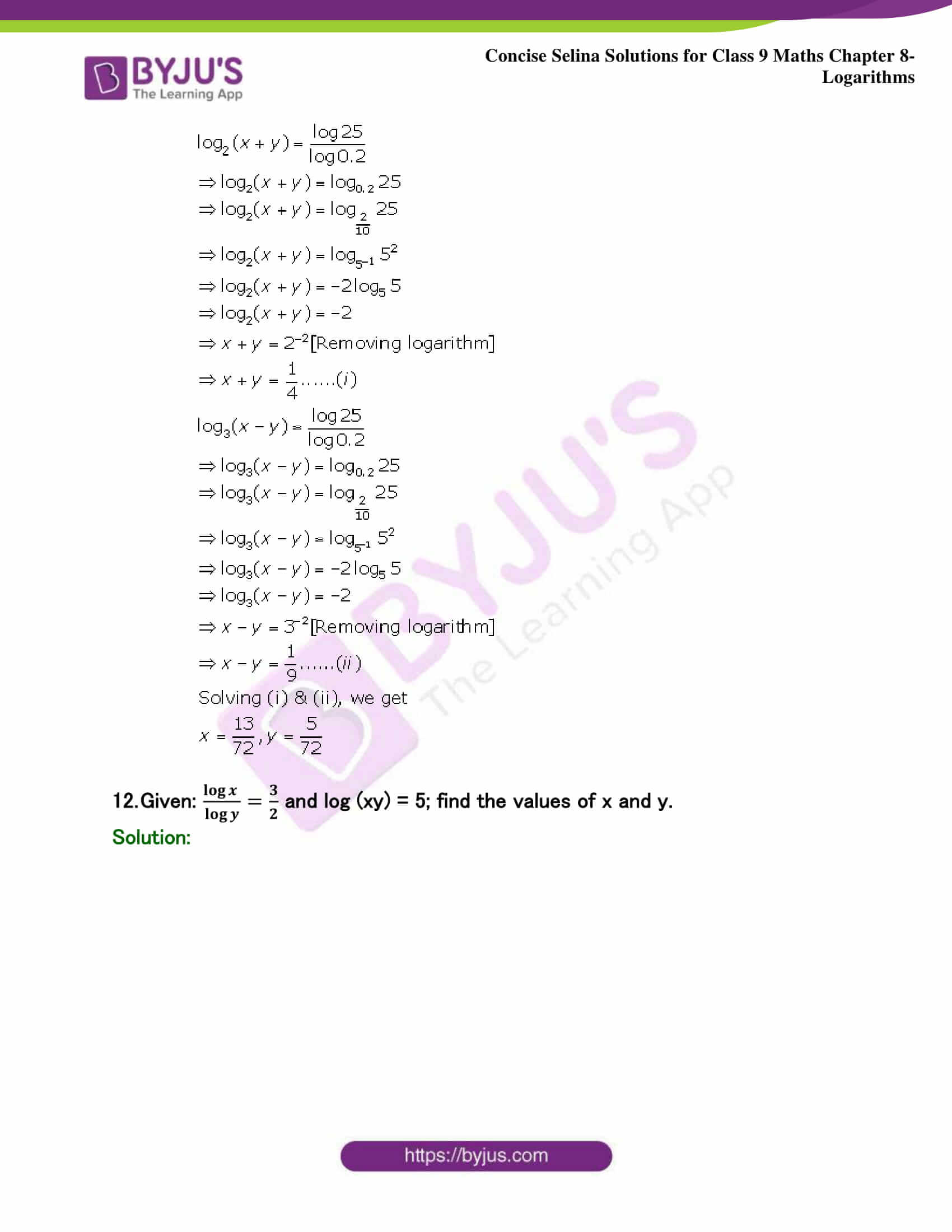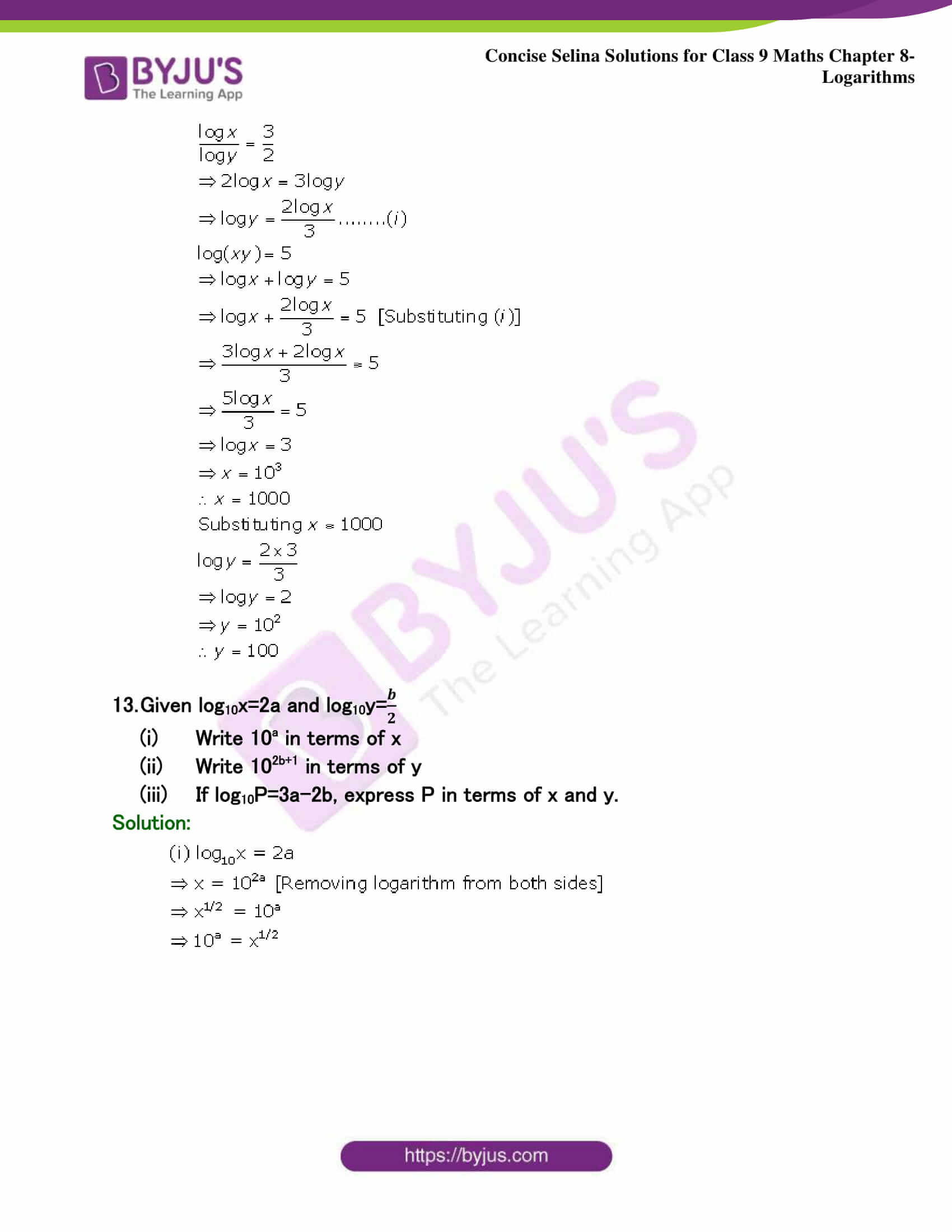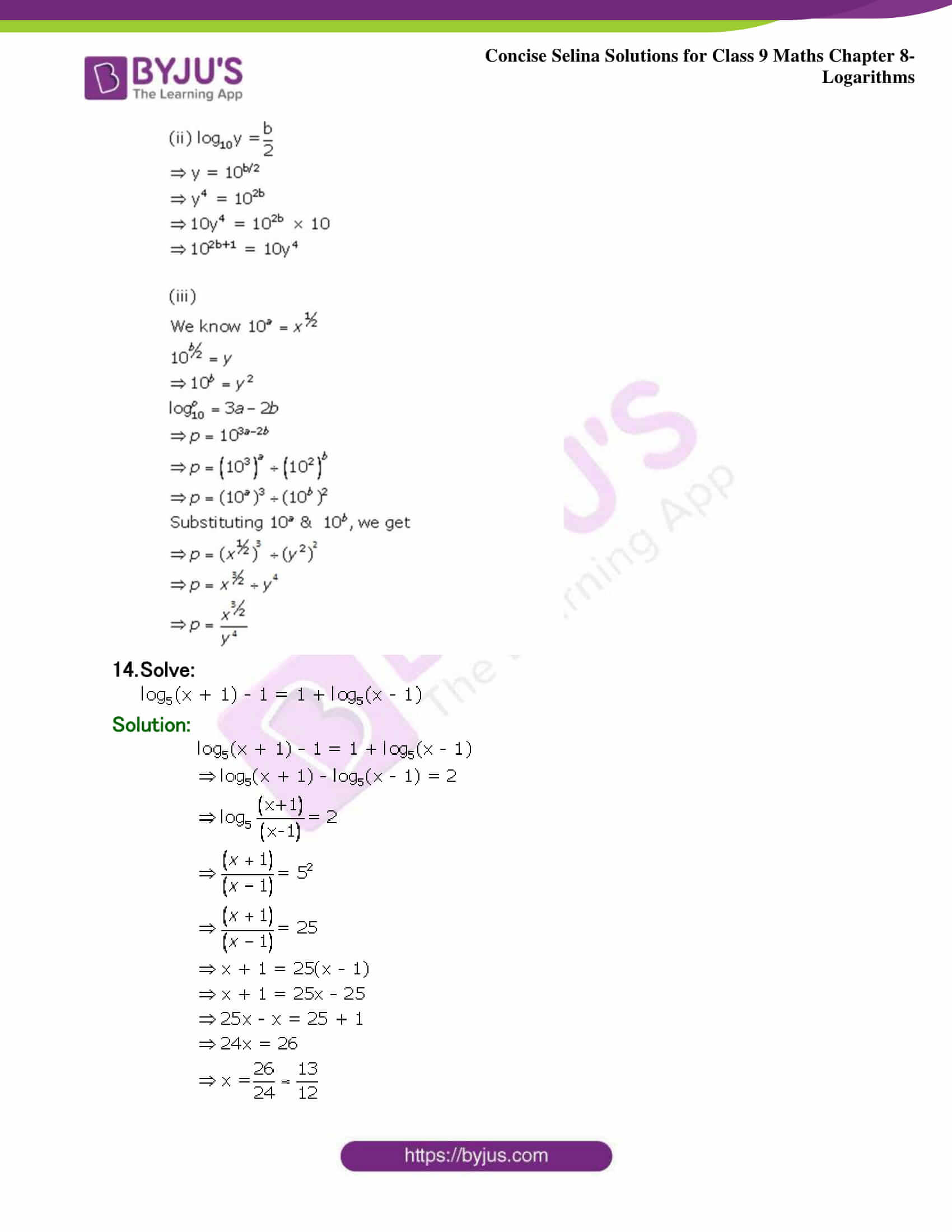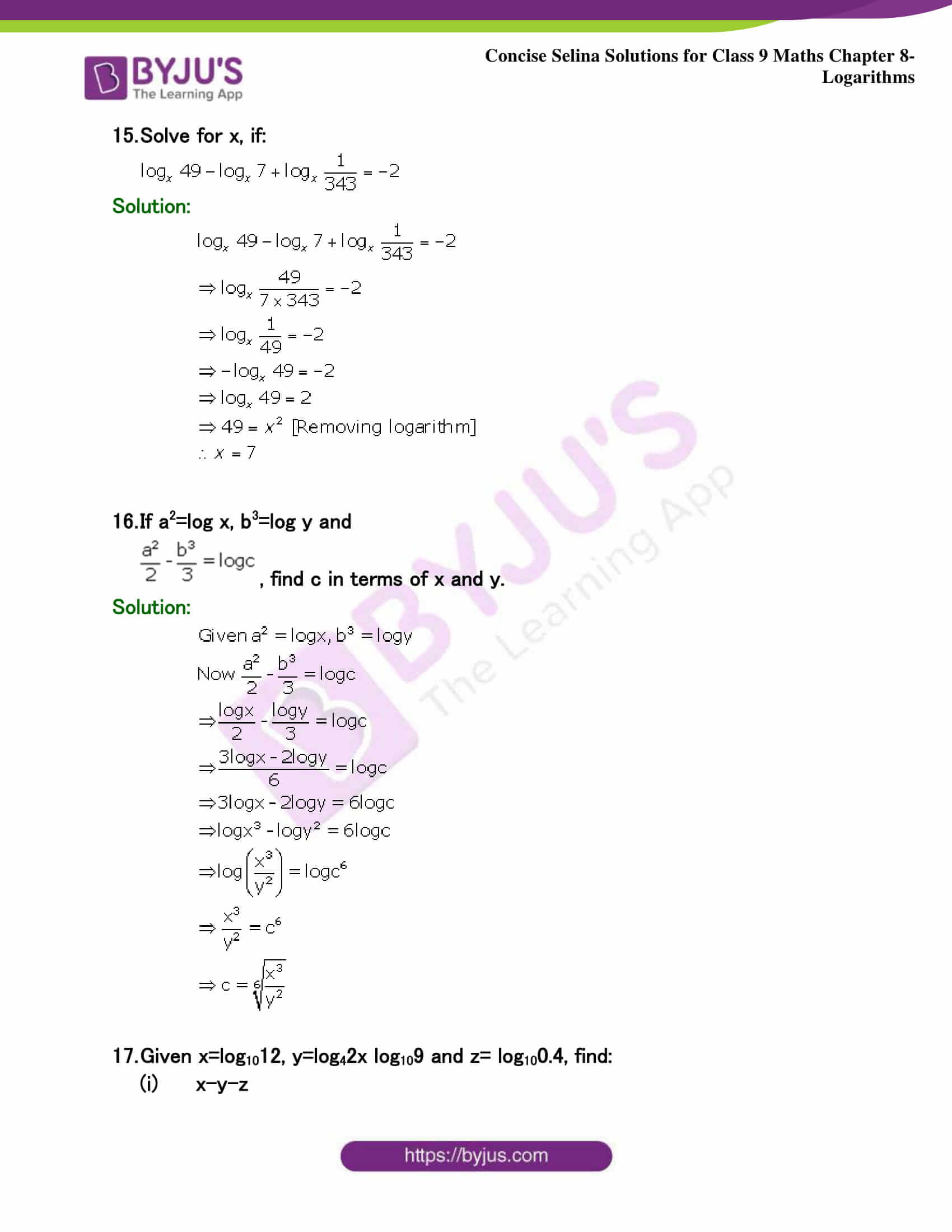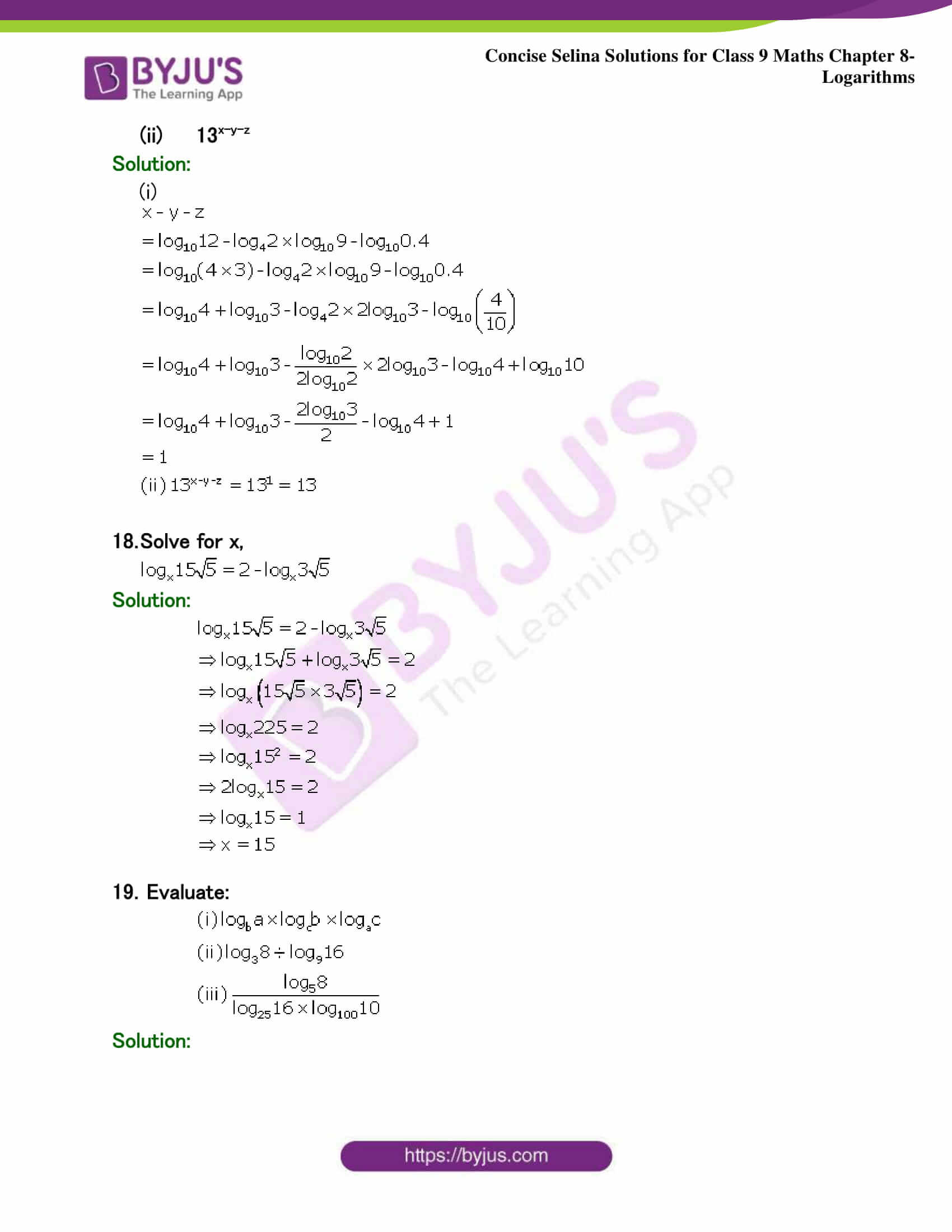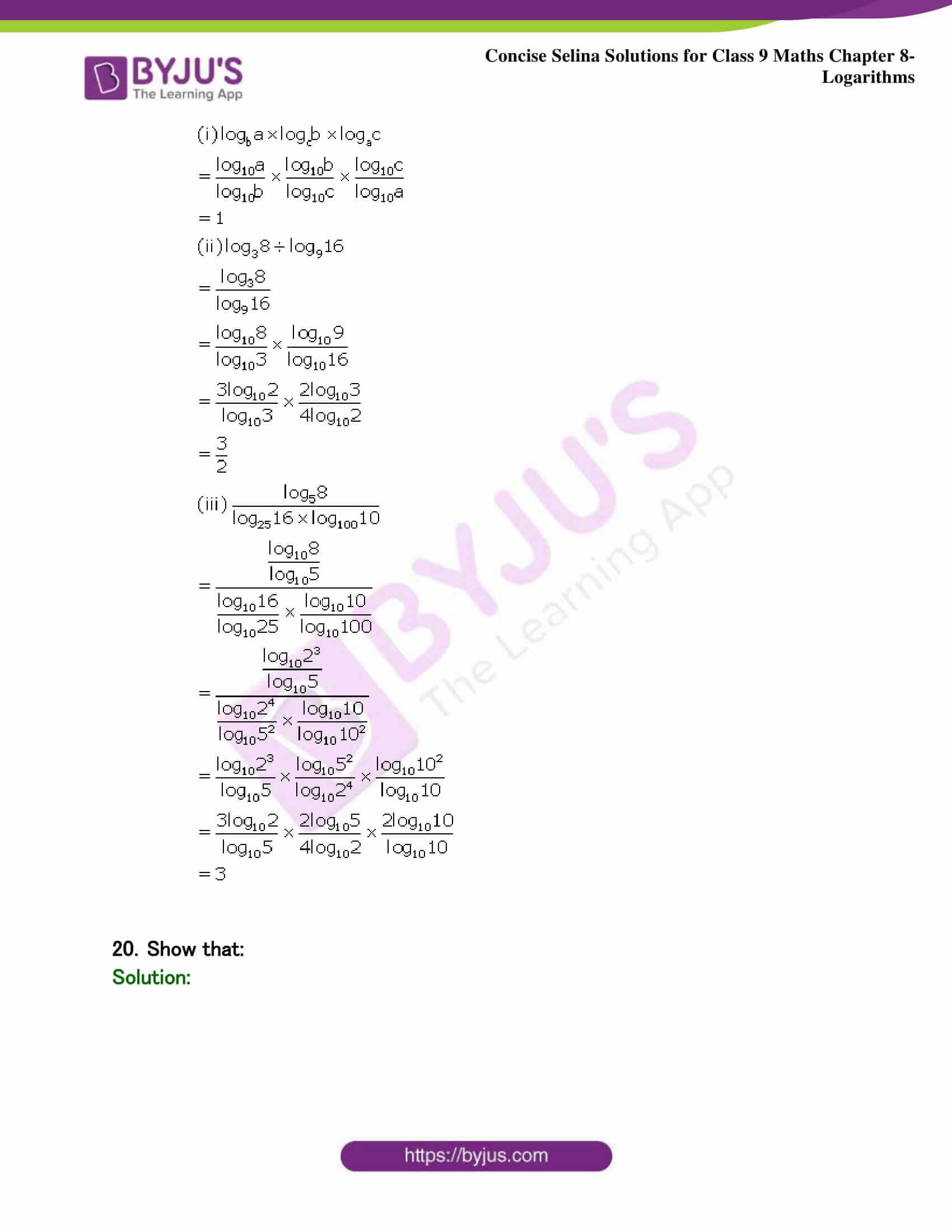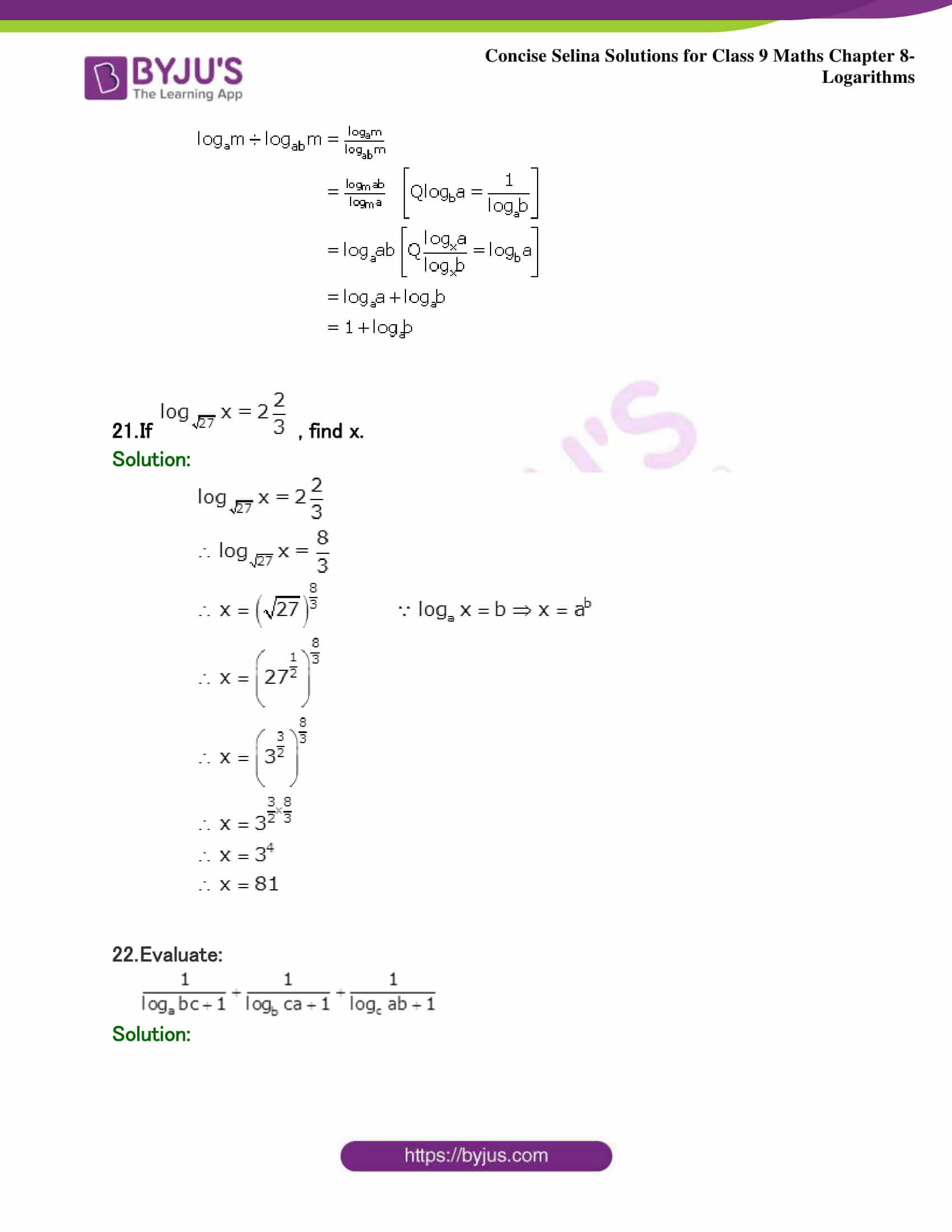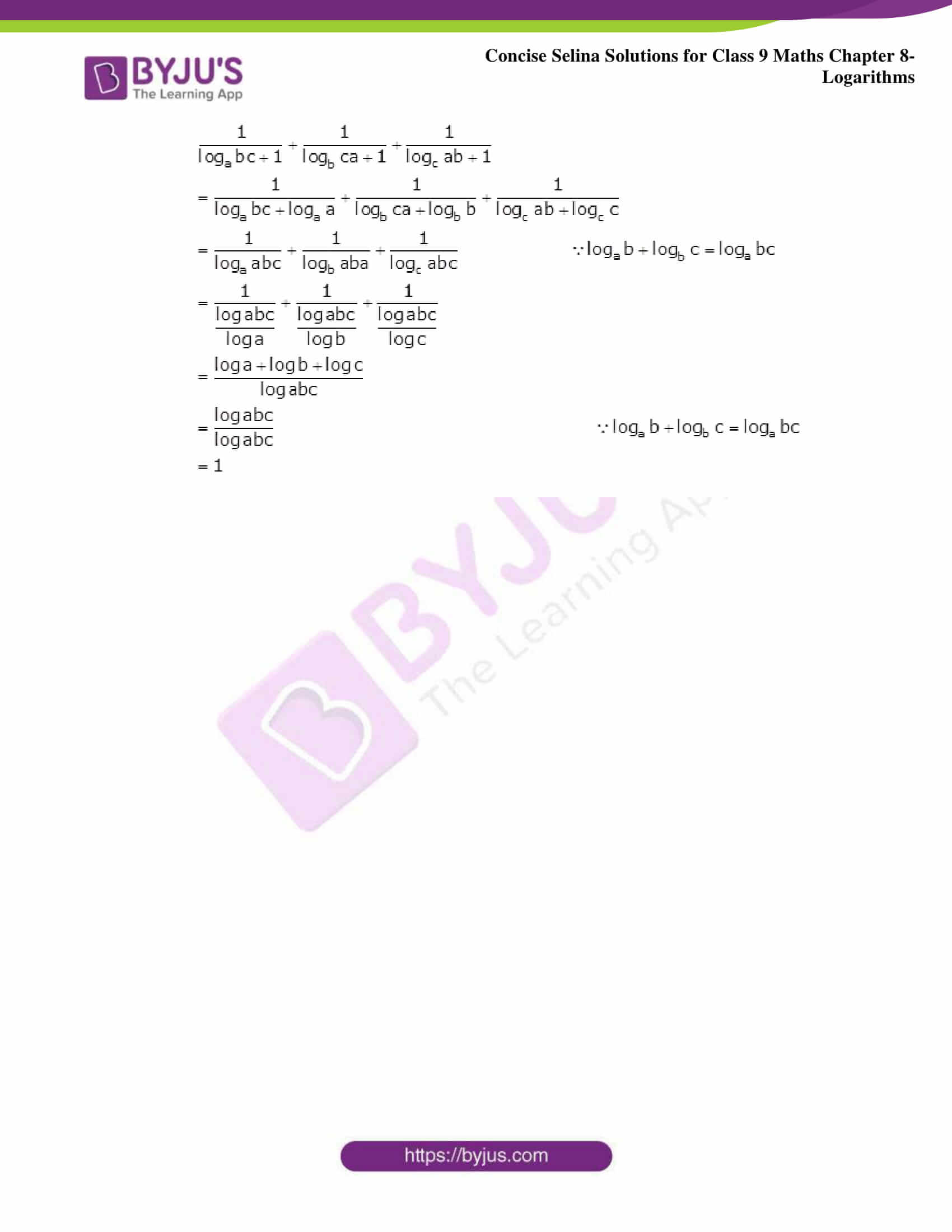## Selina Solutions for Class 9 Maths Chapter 8- Logarithms

The Chapter 8, Logarithms, contains 4 exercises and the Solutions given here contains the answers for all the questions present in these exercises. Let us have a look at some of the topics that are being discussed in this chapter.

8.1 Introduction

8.2 Interchanging

8.3 Laws of logarithms with use

8.4 Expansion of expressions with the help of laws of logarithms